Amin
Topic Author
Posts: 2411
Joined: July 14th, 2002, 3:00 am

### Re: Breakthrough in the theory of stochastic differential equations and their simulation

Friends, mind control agents are getting very impatient to retard me and stop me from my research. I went to a restaurant around noon to eat food. I was not taking it extremely seriously and went to a random restaurant in Wapda town which is a neighborhood close to where I live. As it turned out, army was tracking my movements and they had already reached the restaurant. When I took first bite of food, I realized that it was drugged. I did not have anything other than just one bite and I left the restaurant. Several hours later, I am still under hangover from that bite despite that I had good food somewhere else and also drank a good red bull. I really remained unable to think properly for several long hours despite that I had taken only one bite of bad drugged food.
I also noticed today that they had planted some device in my motorbike seat to charge my back. They had not done this for several months and it was only today that I noticed it again. One reason might be that I went for a walk early in the morning and was determined to continue my morning walk everyday and reduce my weight. Morning walk not only helps keep me physically fit, it also helps to connect the brain in new ways and keeps it thinking intelligently reducing the effect of mind control. It is my guess that mind control agents realized that I might get out of their whatever control they have due to my walk and they needed to add other things to mind control and decided to plant devices in motorbike seat so that they could continue to charge my back and make their mind control very effective.
I want to request friends to please protest to American government to ask pentagon generals to end my mind control and let me live with my human dignity intact. Somebody has to teach humanity to crooks of pentagon that they first need to respect human beings before getting promoted. These anal crooks of pentagon have no regard for any human ethics or good reputation associated with American people and they are simply dying to enter the paradise of billionaire Goldfather and have become instruments of Goldfather to retard anyone Goldfather wishes and these lowly crooks of pentagon are very willing to destroy the great reputation associated with American nation so that they could enter the paradise of billionaire goldfather.
You think life is a secret, Life is only love of flying, It has seen many ups and downs, But it likes travel more than the destination. Allama IqbalAmin
Topic Author
Posts: 2411
Joined: July 14th, 2002, 3:00 am

### Re: Breakthrough in the theory of stochastic differential equations and their simulation

Friends, I have been able to greatly improve my previous regression program. I have mostly been able to get good shapes for densities that have as few as only ten data observations and do all sorts of hermite regressions on these local densities capturing non-linear effects. I have especially improved the program that converted the data to its standard Z-values based on the parameters of the Z-series and now it is very rare to get a Z-value that has a residual with data given the parameters of the Z-series. I have also written a program for multivariable, and multi-hermite regressions in which you have to only input a matrix of explanatory variables and the vector of regressand and it will return the coefficients of regressions for hermites associated with various variables right away. I hope to post the program later today but it can still possibly be another day since I still have to check a few things. It is very interesting how hermite polynomials capture the non-linear effects in the data. I look forward to posting the new improved programs in a day.
You think life is a secret, Life is only love of flying, It has seen many ups and downs, But it likes travel more than the destination. Allama IqbalAmin
Topic Author
Posts: 2411
Joined: July 14th, 2002, 3:00 am

### Re: Breakthrough in the theory of stochastic differential equations and their simulation

Friends, I am working on multivariate regression program. I regresses Apple stock returns for twenty days on Microsoft stock returns and Nvidia stock returns. No financial significance but interesting to be able to do a good regression in multiple hermite polynomial framework. R-Squared for this regression is 0.77 . Here is a quick 2D graph rotated to show how the regression surface is passing through the scatterplot. There were only twenty data days in regression.
.
.This is another view of the same graph.
.
.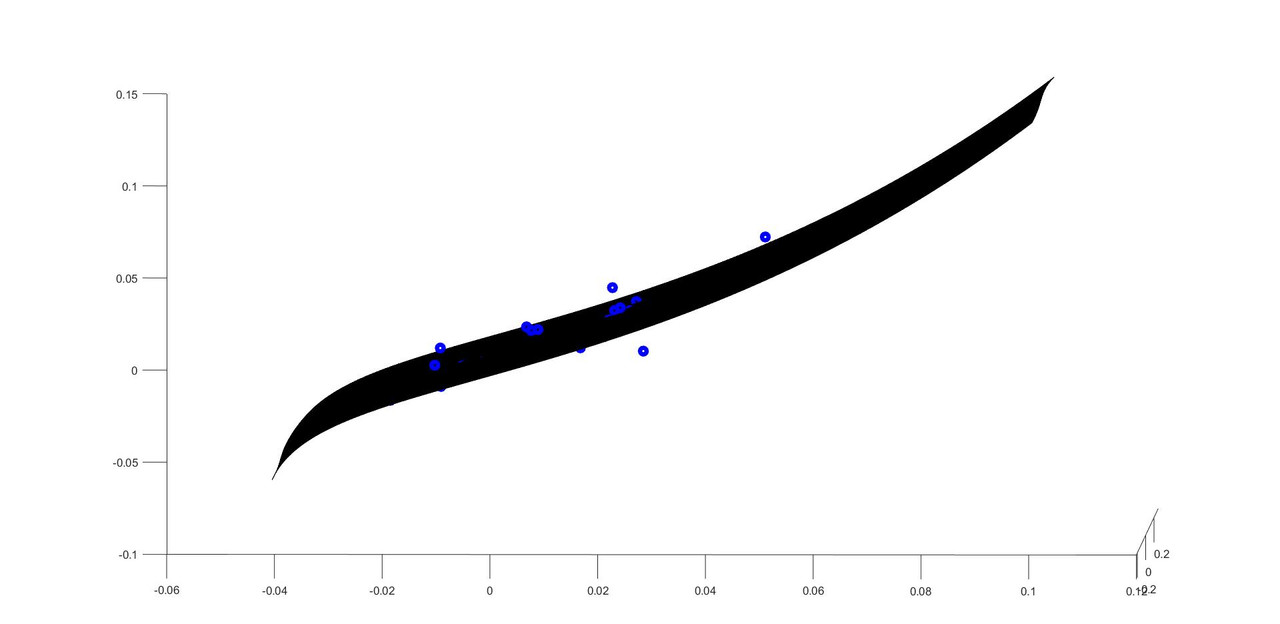You think life is a secret, Life is only love of flying, It has seen many ups and downs, But it likes travel more than the destination. Allama IqbalAmin
Topic Author
Posts: 2411
Joined: July 14th, 2002, 3:00 am

### Re: Breakthrough in the theory of stochastic differential equations and their simulation

Friends, though my program is complete, I still have to add comments and write equation numbers on code that could be related to latex equations later explained on the forum. I also want to review the code if it can be revised to become easier and more understandable. Therefore I will be posting my code later tomorrow and then also explain the logic with latex equations. Sorry for this delay but we are already there and I hope friends would like my multivariate regressions with multiple hermite polynomials code tomorrow.
You think life is a secret, Life is only love of flying, It has seen many ups and downs, But it likes travel more than the destination. Allama IqbalAmin
Topic Author
Posts: 2411
Joined: July 14th, 2002, 3:00 am

### Re: Breakthrough in the theory of stochastic differential equations and their simulation

Friends, I have flu today and very difficult to work. My nose is running like anything so I am posting the program and will give explanation later.
here are the matlab files for multivariate multiple hermite polynomial regression.
I have shown the user density of each variable involved in regression. Many times for smaller samples, these densities are not stable and the first derivative of Z-series variable changes sign and density folds backwards on itself at the ends instead of having deep tails. Usually this problem occurs when fourth moment calculated from small samples is smaller than actual raw fourth moment (even though raw sample moments are unbiased predictors of true raw moments). In my density calculation, I have increased fourth raw moment by a small value while keeping first three moments at their sample values. This very heuristic correction in fourth raw moment is larger for very small samples and negligible for larger samples. I will continue to work more systematically on proper density construction from small sample data after posting this program. But this heuristic correction really helps to construct more stable densities with better regression results. But this has to be more systematically studies.
Here is how I made change to fourth raw moments that is larger for small samples and negligible for larger samples.
rMu(4)=sum(DPrices(1:NDays0).^4)/NDays0*(NDays0)*(NDays0)/(NDays0-1)/(NDays0-1.5);
So the fourth raw moment is increased by   (NDays0)*(NDays0)/(NDays0-1)/(NDays0-1.5)
This is totally heuristic. I wanted to complete a working version of the program first and then later come back to details that need to be fixed properly and rigorously.

All you have to do is to visualize the marginal density of each variable and if it is fine in the tails, your regression would be good. Also because in new program, when densities are good enough in tail, you will not encounter any problems finding standardized Z-values of each random variable.
There is a density visualization program but that will work only for two regressors. For more regressors, you will have to alter the program for visualization purposes.
Here are the program files.
.
.
function [] = MultivariableMultiHermiteRegressionExample()

%Below, read AAPL, AMZN, GOOGL,MSFT,NVDA, NASDAQ100 daily open and
%close data files and extract daily open from the files. All files are
%exactly the same length and therefore corresponding array entries are
%from the same dates.
[NDays,NVar]=size(T);
ApplPrices(1:NDays)=table2array(T(1:NDays,2));
AmznPrices(1:NDays)=table2array(T(1:NDays,2));
GooglPrices(1:NDays)=table2array(T(1:NDays,2));
MsftPrices(1:NDays)=table2array(T(1:NDays,2));
NvdaPrices(1:NDays)=table2array(T(1:NDays,2));
NasdaqPrices(1:NDays)=table2array(T(1:NDays,2));

StartI=01;%choose the start of the regression
EndI=46;%choose the last entry of the regression
NDays=EndI-StartI;

%Below calculate daily log-returns for regression.
DApplPrices(1:NDays)=log(ApplPrices(StartI+1:EndI))-log(ApplPrices(StartI:EndI-1));
DMsftPrices(1:NDays)=log(MsftPrices(StartI+1:EndI))-log(MsftPrices(StartI:EndI-1));
DNvdaPrices(1:NDays)=log(NvdaPrices(StartI+1:EndI))-log(NvdaPrices(StartI:EndI-1));
clf;
Y=DApplPrices(1:NDays); %Y is regressand
X(1,1:NDays)=DMsftPrices(1:NDays); %First row containing first explnatory variable
X(2,1:NDays)=DNvdaPrices(1:NDays); %Second row containing first explnatory variable
MM=2;   %Number of explanatory variables
TT=NDays;%Number of Data Entries in regression
HH=3; %Number of hermite polynomils used in regression
DoMultivariableMultiHermiteRegression(Y,X,MM,TT,HH) %Call the function that does regression.

end
.
.
.
function []= DoMultivariableMultiHermiteRegression(Y1,X1,MM,TT,HH)

W(1:MM,1:TT)=X1(1:MM,1:TT); %We add regressors to W
W(MM+1,1:TT)=Y1(1,1:TT);% We add regressand to W
%Above we added all explanatory and explained variables in a single matrix
%so that we could calculate correlations between respective Z-values of the
%W matrix.

for mm=1:MM+1  %total number of variables is number of regressors + 1(which is regressand)
X(1:TT)=W(mm,1:TT); %Extract the time series of each variable in W
rMu(mm,:) = CalculateFourRawMomentsFromDataA(X,TT);%Calculate four raw moments of each time series.
[c0(mm),c(mm,:)] = CalculateZSeriesDensityFromRawMomentsM4(rMu(mm,:));%Calculate Z-series from each moment
PlotZSeriesDensity(c0(mm),c(mm,:),'g');%Look at the density to make sure it is valid. If the tails fall
%backwards in either direction, regression result can be flawed.
Z(mm,1:TT)=CalculateZgivenXAndZSeriesC3(X,c0(mm),c(mm,:));%Calculate Z for each data entry in time series of every variable(one by one through loop)
[ch0(mm),ch(mm,:)]=ConvertZCoeffsToHCoeffs(c0(mm),c(mm,:),3);%Convert the Z-coeffs to H-coeffs

end

%below we calculate regression coefficients between standardized Z-values
%of regressors and regressand. We only use correlations and covariances do
%not enter this calculation.

NN=MM+1; %This is regressors + 1 which is explained variable.
%Z
%str=input('Look at Z');
%Z is a matrix containing standardized Z's of both regressors and
%regressand and we calculate all cross correlations in it.Three different
%correlation matrices are calculated each for 1st, second and third hermite
%as we are using three hermites in our analysis.
CorrH = CalculateCorrelationMatricesHermiteCH(Z,NN,TT,HH)
str=input('Look at CorrH');
for hh=1:HH %Loop over all hermite polynomials and calculate regression coefficients between
%standardized Z-values of observation.
CorrHR(1:MM,1:MM)=CorrH(1:MM,1:MM,hh);%Correlation matrix containing regressors
CorrHr(1:MM)=CorrH(NN,1:MM,hh);%Correlation vector containing correlations between regressors and regressand
BetaHr(1:MM,hh)=CorrHr/CorrHR;%Do a inv(correlation matrix) right multiply to calculate regression coefficients between standardized data
%This is repeated for each hermiote.
%BetaHr(mm,hh) is regression coefficient from mmth standardized explanatory
%variable in case of hhth hermite regression.
end

%If there are two explanatory variables they can also be visualized as
%below. The visualization function below will work only in case of two explanatory
%variables.
if(MM==2)
CalculateAndPlotRegressandVsTwoRegressors(MM,ch0,ch,BetaHr,X1,Y1,TT);
end

%Below we calculate predicted value of each data point and then calculate
%The code block below assumes we are using three hermites in calculations
%and you will have to slightly alter it for different number of hermits.

TSS=0;
ESS=0;
for tt=1:TT
Yreg(tt)=ch0(NN);
for mm=1:MM
Yreg(tt)=Yreg(tt)+ch(NN,1).*BetaHr(mm,1)*Z(mm,tt)+ch(NN,2).*BetaHr(mm,2)*(Z(mm,tt).^2-1)+ ...
ch(NN,3).*BetaHr(mm,3)*(Z(mm,tt).^3-3*Z(mm,tt));
%Add a fourth hermite term if there are four hermites in regression.
end
TSS=TSS+(Y1(tt)-ch0(NN)).^2;  %Observed difference from mean.Squared.
ESS=ESS+(Yreg(tt)-ch0(NN)).^2;
end

TSS
ESS
Rsquared
str=input('Look at Regression numbers');

end


.
.
.
function [rMu] = CalculateFourRawMomentsFromDataA(DPrices,NDays0)

rMu(1)=sum(DPrices(1:NDays0))/NDays0;
rMu(2)=sum(DPrices(1:NDays0).^2)/(NDays0);
rMu(3)=sum(DPrices(1:NDays0).^3)/NDays0;%*(NDays0)/(NDays0-1);
rMu(4)=sum(DPrices(1:NDays0).^4)/NDays0*(NDays0)*(NDays0)/(NDays0-1)/(NDays0-1.5);
%rMu(4)=sum(DPrices(1:NDays0).^4)/NDays0*(NDays0)/(NDays0-1);

end


.
.
.
function [c0,c] = CalculateZSeriesDensityFromRawMomentsM4(rMu)

mOrder=4;
[Mu1,cMu] = ConvertRawMomentsToCentralMoments(rMu,mOrder);

sMu(1)=0;
sMu(2)=1;
sMu(3)=cMu(3)/cMu(2).^1.5;
sMu(4)=cMu(4)/cMu(2).^2.0;
%sMu(5)=cMu(5)/cMu(2).^2.5;
%sMu(6)=cMu(6)/cMu(2).^3.0;
%sMu(7)=cMu(7)/cMu(2).^3.5;
%sMu(8)=cMu(8)/cMu(2).^4.0;

iter=20;
bGuess(1:3)=0;
bGuess(1)=1;

SeriesOrder=3;
NMoments=4;

[F,dF] = CalculateMomentsAndDerivatives_0(sMu,c0,c,SeriesOrder,SeriesOrder,NMoments);

da(1,1)=c0;
da(2:SeriesOrder+1,1)=c(1:SeriesOrder);

[Moments] = CalculateMomentsOfZSeries(c0,c,SeriesOrder,NMoments);

%Replace with your own more intelligent objective function if you like.
ObjBest=100000*(abs(sMu(1)-Moments(1)))+abs(sMu(2)-Moments(2))+abs((sMu(3)-Moments(3))^(1/1.5))+abs((sMu(4)-Moments(4))^(1/2.0));

b0Best=c0;
bBest(1:SeriesOrder)=c(1:SeriesOrder);

nn=0;
while((nn<20)&&((abs(F(1,1))>.000000000001) || (abs(F(2,1))>.000000000001) || (abs(F(3,1))>.000000000001) || (abs(F(4,1))>.00000000001)  ))

nn=nn+1;
%Below Newton matrix equation to improve the Z-series coefficients guess at previous step.
da=da-dF\F;

%b0=median
b0=da(1,1);
b(1:SeriesOrder)=da(2:SeriesOrder+1,1);

%[F,dF] = CalculateCumulantsAndDerivativesFromMoments_0(C,b0,b,SeriesOrder,SeriesOrder,NoOfCumulants);
[F,dF] = CalculateMomentsAndDerivatives_0(sMu,b0,b,SeriesOrder,SeriesOrder,NMoments);
[IsValidFlag] =1;% CheckIsValidDensity(b0,b);
[Moments] = CalculateMomentsOfZSeries(b0,b,SeriesOrder,NMoments);

ObjNew=100000*(abs(sMu(1)-Moments(1)))+abs(sMu(2)-Moments(2))+abs((sMu(3)-Moments(3))^(1/1.5))+abs((sMu(4)-Moments(4))^(1/2.0));

if((ObjBest>ObjNew) &&( IsValidFlag))

ObjBest=ObjNew;
b0Best=b0;
bBest(1:SeriesOrder)=b(1:SeriesOrder);
end

da(1,1)=b0;
da(2:SeriesOrder+1,1)=b(1:SeriesOrder);

end
c0=b0Best;%Best;
c(1:SeriesOrder)=bBest;%Best(1:SeriesOrder);

c0=c0*sqrt(cMu(2));
c=c*sqrt(cMu(2));

c0=c0+Mu1;

[FittedMoments] = CalculateMomentsOfZSeries(c0,c,SeriesOrder,NMoments);
%%%%%%%%%%%%%%%%%%%%%%%%%%%%%%%%%%%%%%%%%%%%%%%%%%%%%%%%%%%%%%%%%%%%%%%%%%%%%%%%%%%%%%%%%%%%%%
%Please Uncomment this if you want to see a comparison of input moments and
%fitted moments
rMu
FittedMoments
str=input('Look at comparison of fitted moments with input moments');
%%%%%%%%%%%%%%%%%%%%%%%%%%%%%%%%%%%%%%%%%%%%%%%%%%%%%%%%%%%%%%%%%%%%%%%%%%%%%%%%%%%%%%%%%%%%%5
%COMMENT lines below if you do not want to see the density of new Z-series random variable with
%fitted moments.
%First calculate normal random variable on a grid below
dNn=.1/2;   % Normal density subdivisions width. would change with number of subdivisions
Nn=45*4;  % No of normal density subdivisions
NnMid=((1+Nn)/2)*dNn;
Z(1:Nn)=(((1:Nn)*dNn)-NnMid);
%Z
%str=input('Look at Z');
%Now calculate Y as a function of normal random variable.
Y(1:Nn)=c0;
for nn=1:3
Y(1:Nn)=Y(1:Nn)+c(nn)*Z(1:Nn).^nn;
end

%Now take change of densities derivative of Y with respect to normal
DfY(1:Nn)=0;
for nn=2:Nn-1
DfY(nn) = (Y(nn + 1) - Y(nn - 1))/(Z(nn + 1) - Z(nn - 1));
%Change of variable derivative for densities
end
DfY(Nn)=DfY(Nn-1);
DfY(1)=DfY(2);

%Now calculate the density of Y from density of normal random variable
%using change of probability derivative.
pY(1:Nn)=0;
for nn = 1:Nn
pY(nn) = (normpdf(Z(nn),0, 1))/abs(DfY(nn));
end

plot(Y(1:Nn),pY(1:Nn),'b');
title('Density of the Z-Series Variable with Fitted Moments');

%str=input('Look at the density of Z-series random variable')
%%%%%%%%%%%%%%%%%%%%%%%%%%%%%%%%%%%%%%%%%%%%%%%%%%%%%%%%%%%%%%%%%%%%%%%%%%%%%%%%%%%%%%%%%%%%

end


.
.
.
function [mu1,cMu] = ConvertRawMomentsToCentralMoments(Mu,mOrder)

%cu
%mu
% %mOrder
%str=input('Look at numbers');
mu1=Mu(1);
for mm=2:mOrder
cMu(mm)=0;
for jj=0:mm
if(jj==0)
cMu(mm)=cMu(mm)+ (-1)^(mm-jj).*factorial(mm)/factorial(jj)/factorial(mm-jj)*1*mu1.^(mm-jj);
else
cMu(mm)=cMu(mm)+ (-1)^(mm-jj).*factorial(mm)/factorial(jj)/factorial(mm-jj)*Mu(jj)*mu1.^(mm-jj);
end
end
end


.
.
.
function [c0,c] = PreSmoothingGuessAdvancedFromGuessBestNewC3(cmu,cin,iter)

%Mul=1.0;
SeriesOrder=3;
NMoments=4;

EZ(1)=0;
EZ(2)=1;
for nn=3:NMoments*SeriesOrder+SeriesOrder+2
if rem(nn,2)==1
EZ(nn)=0;
else
EZ(nn)=EZ(nn-2)*(nn-1);
EZ(nn);
end
end

c0=-(cin(2));  %This is for zero mean condition.
c=cin;
MaxIter=10;

for nn=1:iter     %increase the iterations over coefficients if neeeded

%%%%%%%%%%%%%%%%%%%%%%%%%%%%%%%%%%%%%%%%%%%%%%%%%%%%%%%%%

[M3,dc2] = CalculateParticularMomentAndDerivativeOfItsCoeff(c0,c,SeriesOrder,3,EZ);

tolerance=.0000001*cmu(3);
mm=0;
while ((abs(M3-cmu(3)) >tolerance ) && (mm<MaxIter))
mm=mm+1;
c(2)=c(2)-(M3-cmu(3))/dc2;

[M3,dc2] = CalculateParticularMomentAndDerivativeOfItsCoeff(c0,c,SeriesOrder,3,EZ);

end

c0=-(c(2));

[SecondMoment] = CalculateSecondMomentC3(c0,c);

c=c/sqrt(SecondMoment);
c0=c0/sqrt(SecondMoment);

%[c0,c] = IterateSmoothingGuessOverPreviousMoments(cmu,c,3,2,EZ);

%%%%%%%%%%%%%%%%%%%%%%%%%%%%%%%%%%%%%%%%%%%%%%%%%%%%%%%%%%5
[M4,dc3] = CalculateParticularMomentAndDerivativeOfItsCoeff(c0,c,SeriesOrder,4,EZ);

tolerance=.0000001*cmu(4);
mm=0;
while ((abs(M4-cmu(4)) >tolerance ) && (mm<MaxIter))
mm=mm+1;
c(3)=c(3)-(M4-cmu(4))/dc3;

[M4,dc3] = CalculateParticularMomentAndDerivativeOfItsCoeff(c0,c,SeriesOrder,4,EZ);

end

[SecondMoment] = CalculateSecondMomentC3(c0,c);

c=c/sqrt(SecondMoment);
c0=c0/sqrt(SecondMoment);

%[c0,c] = IterateSmoothingGuessOverPreviousMoments(cmu,c,4,2,EZ);

%%%%%%%%%%%%%%%%%%%%%%%%%%%%%%%%%%%%%%%%

end

end


.
.
.
function [F,dF] = CalculateMomentsAndDerivatives_0(Ms,a0,a,SeriesOrder,NZterms,NMoments)
%[F,dF] = CalculateCumulantsAndDerivativesFromMoments(C,a0,a,SeriesOrder,SeriesOrder,NoOfCumulants);

%a(1)=a1;
%a(2)=a2;
%a(3)=a3;
%a(4)=a4;
%a(5)=a5;
%a(6)=a6;
%a(7)=a7;

%aa0=a0;
if(NMoments>8)
a0=-(a(2)+3*a(4)+15*a(6)+105*a(8));% ---1
end
if(NMoments==8)
a0=-(a(2)+3*a(4)+15*a(6));% ---1
end
if(NMoments==6)
a0=-(a(2)+3*a(4));% ---1
end

EZ(1)=0;
EZ(2)=1;
for nn=3:NMoments*SeriesOrder+NZterms+2
if rem(nn,2)==1
EZ(nn)=0;
else
EZ(nn)=EZ(nn-2)*(nn-1);
EZ(nn);
end
end
EZ;

EXZ(1,1)=1;
for pp1=1:NZterms
EXZ(1,pp1+1)=EZ(pp1);
end

a(SeriesOrder+1:NMoments*SeriesOrder+1)=0;
b0=a0;
b=a;

for mm=1:NMoments
if(mm>1)
[b0,b] =SeriesProduct(a0,a,b0,b,SeriesOrder*mm);
b(SeriesOrder*mm+1:NMoments*SeriesOrder+1)=0;
end
% b0
% b
%str=input('Look at numbers')
EXZ(mm+1,1)=b0;
for pp2=1:SeriesOrder*mm

EXZ(mm+1,1)=EXZ(mm+1,1)+b(pp2).*EZ(pp2);
end
for pp1=1:NZterms
EXZ(mm+1,pp1+1)=b0.*EZ(pp1);
for pp2=1:SeriesOrder*mm

EXZ(mm+1,pp1+1)=EXZ(mm+1,pp1+1)+b(pp2).*EZ(pp2+pp1);
end
end
end

%u1=EXZ(2,1);
u2=EXZ(3,1);
u3=EXZ(4,1);
u4=EXZ(5,1);

u1=a0+a(2);
if(SeriesOrder>=4)
u1=a0+a(2)+3*a(4);
end
if(SeriesOrder>=6)
u1=u1+15*a(6);
end
if(SeriesOrder>=8)
u1=u1+105*a(8);
end

%k2=u2-u1^2;
%k3=u3-3*u2*u1+2*u1^3;
%k4=u4-4*u3*u1-3*u2^2+12*u2*u1^2-6*u1^4;

du1(1)=1;%----2
% du1(2)=0;%----2
% du1(3)=1;%----2
% du1(4)=0;%----2
% du1(5)=1;%----2
% du1(6)=0;%----2
% du1(7)=15;%----2
% du1(8)=0;%----2
% du1(9)=105;%----2
% du1(10)=0;%----2
du2(1)=2*EXZ(2,1);
du3(1)=3*EXZ(3,1);
du4(1)=4*EXZ(4,1);

%du1(1)=1;%----2
%du2(1)=0;
%du3(1)=0;
%du4(1)=0;

for mm=2:SeriesOrder+1
du1(mm)=EXZ(1,mm);
du2(mm)=2*EXZ(2,mm);
du3(mm)=3*EXZ(3,mm);
du4(mm)=4*EXZ(4,mm);

end

if(NMoments>=5)
u5=EXZ(6,1);

%du5(1)=5*EXZ(5,1);

for mm=1:SeriesOrder+1
du5(mm)=5*EXZ(5,mm);
end

end

if(NMoments>=6)
u6=EXZ(7,1);
%du6(1)=0;

for mm=1:SeriesOrder+1
du6(mm)=6*EXZ(6,mm);
end

end

if(NMoments>=7)
u7=EXZ(8,1);
%str=input('Look at k7 and k71')

%du7(1)=0;

for mm=1:SeriesOrder+1
du7(mm)=7*EXZ(7,mm);
end

end
if(NMoments>=8)

u8=EXZ(9,1);
for mm=1:SeriesOrder+1
du8(mm)=8*EXZ(8,mm);
end

end

if(NMoments>=9)

u9=EXZ(10,1);
for mm=1:SeriesOrder+1
du9(mm)=9*EXZ(9,mm);
end

end
if(NMoments>=10)

u10=EXZ(11,1);
for mm=1:SeriesOrder+1
du10(mm)=10*EXZ(10,mm);
end

end

F(1,1)=u1-Ms(1);
F(2,1)=u2-Ms(2);
F(3,1)=u3-Ms(3);
F(4,1)=u4-Ms(4);
if(NMoments>=5)
F(5,1)=u5-Ms(5);
end
if(NMoments>=6)
F(6,1)=u6-Ms(6);
end
if(NMoments>=7)
F(7,1)=u7-Ms(7);
end
if(NMoments>=8)
F(8,1)=u8-Ms(8);
end
if(NMoments>=9)
F(9,1)=u9-Ms(9);
end
if(NMoments>=10)
F(10,1)=u10-Ms(10);
end

for mm=1:SeriesOrder+1
dF(1,mm)=du1(mm);
dF(2,mm)=du2(mm);
dF(3,mm)=du3(mm);
dF(4,mm)=du4(mm);
if(NMoments>=5)
dF(5,mm)=du5(mm);
end
if(NMoments>=6)
dF(6,mm)=du6(mm);
end
if(NMoments>=7)
dF(7,mm)=du7(mm);
end
if(NMoments>=8)
dF(8,mm)=du8(mm);
end
if(NMoments>=9)
dF(9,mm)=du9(mm);
end
if(NMoments>=10)
dF(10,mm)=du10(mm);
end
end

end


.
.
.
function [Moments] = CalculateMomentsOfZSeries(a0,a,SeriesOrder,NMoments)

%aa0=a0;
%a0=0;% ---1

EZ(1)=0;
EZ(2)=1;
%LogEZ(2)=0;
for nn=3:NMoments*SeriesOrder
if rem(nn,2)==1
EZ(nn)=0;
else
EZ(nn)=EZ(nn-2)*(nn-1);
%LogEZ(nn)=log(EZ(nn-2))+log(nn-1);
%EZ(nn);
end
end
%EZ
%EZ(1:30)
%str=input('Look at numbers')
a(SeriesOrder+1:SeriesOrder*NMoments+1)=0;
b0=a0;
b=a;

for mm=1:NMoments
if(mm>1)
%[b0,b] =SeriesProductLogarithmic(a0,a,b0,b,SeriesOrder*mm);

[b0,b] =SeriesProduct(a0,a,b0,b,SeriesOrder*mm);

%b0-bb0

%b-bb

%str=input('Look at two products')

b(SeriesOrder*mm+1:SeriesOrder*NMoments+1)=0;
end
% Logb=log(abs(b));
% Signb=sign(b);
EXZ(mm,1)=b0;
for pp2=1:SeriesOrder*mm
if rem(pp2,2)==0
%EXZ(mm,1)=EXZ(mm,1)+exp(Logb(pp2)+LogEZ(pp2)).*Signb(pp2);
EXZ(mm,1)=EXZ(mm,1)+b(pp2).*EZ(pp2);
%           b(pp2).*EZ(pp2)
%           exp(Logb(pp2)+LogEZ(pp2)).*Signb(pp2)
%           mm
%           pp2
%str=input('Look at moment values')
end
end
end

for nn=1:NMoments
Moments(nn)=EXZ(nn,1);
end

end


.
.
.
function [EnMoment,dEnMomentdCoeff] = CalculateParticularMomentAndDerivativeOfItsCoeff(a0,a,SeriesOrder,nMoment,EZ)

% if(SeriesOrder>=8)
% a0=-(a(2)+3*a(4)+15*a(6)+105*a(8));% ---1
% end
% if(SeriesOrder<8)
% a0=-(a(2)+3*a(4)+15*a(6));% ---1
% end
%
% EZ(1)=0;
% EZ(2)=1;
% for nn=3:NMoments*SeriesOrder+NZterms+2
%     if rem(nn,2)==1
%         EZ(nn)=0;
%     else
%         EZ(nn)=EZ(nn-2)*(nn-1);
%         EZ(nn);
%     end
% end
% EZ;

%EXZ(1,1)=1;
%for pp1=1:NZterms
%        EXZ(1,pp1+1)=EZ(pp1);
%end

a(SeriesOrder+1:nMoment*SeriesOrder+1+SeriesOrder)=0;
b0=a0;
b=a;

for mm=2:nMoment

[b0,b] =SeriesProduct(a0,a,b0,b,SeriesOrder*mm+SeriesOrder+1);
b(SeriesOrder*mm+1:nMoment*SeriesOrder+SeriesOrder+1)=0;
if(mm==nMoment-1)
dEnMomentdCoeff=b0.*EZ(nMoment-1);
for pp2=1:SeriesOrder*nMoment-1

dEnMomentdCoeff=dEnMomentdCoeff+b(pp2).*EZ(pp2+nMoment-1);
end
dEnMomentdCoeff=dEnMomentdCoeff*nMoment;
end
if(mm==nMoment)
EnMoment=b0;
for pp2=1:SeriesOrder*nMoment
EnMoment=EnMoment+b(pp2).*EZ(pp2);

end
end
end

end


.
.
.
function [SecondMoment] = CalculateSecondMomentC3(a0,a)

SecondMoment=a0^2+a(1)^2 +2* a0* a(2) +3*(a(2).^2+2* a(1).* a(3))+ ...
15*(a(3).^2) ;

end


.
.
.
function [] = PlotZSeriesDensity(c0,c,Color)

Index=length(c);

dNn=.1/2;   % Normal density subdivisions width. would change with number of subdivisions
Nn=45*4;  % No of normal density subdivisions
NnMid=((1+Nn)/2)*dNn;
Z(1:Nn)=(((1:Nn)*dNn)-NnMid);
%Z
%str=input('Look at Z');
%Now calculate Y as a function of normal random variable.
Y(1:Nn)=c0;
for nn=1:Index
Y(1:Nn)=Y(1:Nn)+c(nn)*Z(1:Nn).^nn;
end

%Now take change of densities derivative of Y with respect to normal
DfY(1:Nn)=0;
for nn=2:Nn-1
DfY(nn) = (Y(nn + 1) - Y(nn - 1))/(Z(nn + 1) - Z(nn - 1));
%Change of variable derivative for densities
end
DfY(Nn)=DfY(Nn-1);
DfY(1)=DfY(2);

%Now calculate the density of Y from density of normal random variable
%using change of probability derivative.
pY(1:Nn)=0;
for nn = 1:Nn
pY(nn) = (normpdf(Z(nn),0, 1))/abs(DfY(nn));
end

plot(Y(1:Nn),pY(1:Nn),Color);
title('Density of the Z-Series Variable with Fitted Moments');
str=input('Look at density');

end


.
.
.
function [Z] = CalculateZgivenXAndZSeriesC3(X,c0,c)

Z=(X-c0)/c(1)-(c(2) * (X-c0).^2)/c(1).^3+(((2* c(2).^2)./c(1).^2-c(3)./c(1)).* (X-c0).^3)./c(1).^3;
mm=0;
while((max(abs(X-(c0+c(1)*Z+c(2)*Z.^2+c(3)*Z.^3)))>.00000001) && (mm<=100))

X0=c0+c(1)*Z+c(2)*Z.^2+c(3).*Z.^3;
dX0dZ=c(1)+2.*c(2)*Z+3.*c(3).*Z.^2;
Z=Z-(X0-X)./(dX0dZ);
mm=mm+1;
end

if(mm>=100)
pp=0;
for nn=1:length(X)
if( abs(X(nn)-(c0+c(1)*Z+c(2)*Z.^2+c(3)*Z.^3))>.00000001 )
pp=pp+1;
Y(pp)=X(nn);
Index(pp)=nn;
end
end
for ppp=1:pp
ZZ(ppp)=CalculateZgivenXAndZSeriesBisectionC3(Y(ppp),c0,c);
Z(Index(ppp))=ZZ(ppp);
end
end

end


.
.
.
function [Z]=CalculateZgivenXAndZSeriesBisectionC3(Y,c0,c)

if(Y>c0)
if(Y< c0+c(1)*.5+c(2)*.5^2 +c(3) * .5^3)
Za=0;
Zb=.5;
elseif(Y< c0+c(1)*1+c(2)*1^2 +c(3) * 1^3)
Za=.5;
Zb=1;
elseif(Y< c0+c(1)*1.5+c(2)*1.5^2 +c(3) * 1.5^3)
Za=1;
Zb=1.5;
elseif(Y< c0+c(1)*2+c(2)*2^2 +c(3) * 2^3)
Za=1.5;
Zb=2;
elseif(Y< c0+c(1)*2.5+c(2)*2.5^2 +c(3) * 2.5^3)
Za=2;
Zb=2.5;
elseif(Y< c0+c(1)*3+c(2)*3^2 +c(3) * 3^3)
Za=2.5;
Zb=3;
elseif(Y< c0+c(1)*3.5+c(2)*3.5^2 +c(3) * 3.5^3)
Za=3;
Zb=3.5;
elseif(Y< c0+c(1)*4+c(2)*4^2 +c(3) * 4^3)
Za=3.5;
Zb=4;
elseif(Y< c0+c(1)*4.5+c(2)*4.5^2 +c(3) * 4.5^3)
Za=4;
Zb=4.5;
else
Za=4.5;
Zb=5.5;
end
for mm=1:100
Zmid=.5*Za+.5*Zb;
if(Y< c0+c(1)*Zmid+c(2)*Zmid^2 +c(3) * Zmid^3)
Zb=Zmid;
elseif(Y> c0+c(1)*Zmid+c(2)*Zmid^2 +c(3) * Zmid^3)
Za=Zmid;
end
Z=.5*Za+.5*Zb;
Za
Zb
Z
end

end

if(Y<c0)
if(Y> c0+c(1)*-.5+c(2)*(-.5)^2 +c(3) * (-.5)^3)
Za=-.5;
Zb=0;
elseif(Y> c0+c(1)*(-1)+c(2)*(-1)^2 +c(3) * (-1)^3)
Za=-1;
Zb=-.5;
elseif(Y> c0+c(1)*(-1.5)+c(2)*(-1.5)^2 +c(3) * (-1.5)^3)
Za=-1.5;
Zb=-1;
elseif(Y> c0+c(1)*(-2)+c(2)*(-2)^2 +c(3) * (-2)^3)
Za=-2;
Zb=-1.5;
elseif(Y> c0+c(1)*(-2.5)+c(2)*(-2.5)^2 +c(3) * (-2.5)^3)
Za=-2.5;
Zb=-2.0;
elseif(Y> c0+c(1)*(-3)+c(2)*(-3)^2 +c(3) * (-3)^3)
Za=-3;
Zb=-2.5;
elseif(Y> c0+c(1)*(-3.5)+c(2)*(-3.5)^2 +c(3) * (-3.5)^3)
Za=-3.5;
Zb=-3.0;
elseif(Y> c0+c(1)*(-4)+c(2)*(-4)^2 +c(3) * (-4)^3)
Za=-4;
Zb=-3.5;
elseif(Y> c0+c(1)*(-4.5)+c(2)*(-4.5)^2 +c(3) * (-4.5)^3)
Za=-4.5;
Zb=-4.0;
else
Za=-5.5;
Zb=-4.5;
end
for mm=1:100
Zmid=.5*Za+.5*Zb;
if(Y< c0+c(1)*Zmid+c(2)*Zmid^2 +c(3) * Zmid^3)
Zb=Zmid;
elseif(Y> c0+c(1)*Zmid+c(2)*Zmid^2 +c(3) * Zmid^3)
Za=Zmid;
end
Z=.5*Za+.5*Zb;
Za
Zb
Z
end

end

end


.
.
.
function [aH0,aH] = ConvertZCoeffsToHCoeffs(a0,a,SeriesOrder)

if(SeriesOrder==3)
aH0=a0+a(2);
aH(1)=a(1)+3*a(3);
aH(2)=a(2);
aH(3)=a(3);
end

if(SeriesOrder==4)
aH0=a0+a(2)+3*a(4);
aH(1)=a(1)+3*a(3);
aH(2)=a(2)+6*a(4);
aH(3)=a(3);
aH(4)=a(4);
end

if(SeriesOrder==5)
aH0=a0+a(2)+3*a(4);
aH(1)=a(1)+3*a(3)+15*a(5);
aH(2)=a(2)+6*a(4);
aH(3)=a(3)+10*a(5);
aH(4)=a(4);
aH(5)=a(5);
end

if(SeriesOrder==6)
aH0=a0+a(2)+3*a(4)+15*a(6);
aH(1)=a(1)+3*a(3)+15*a(5);
aH(2)=a(2)+6*a(4)+45*a(6);
aH(3)=a(3)+10*a(5);
aH(4)=a(4)+15*a(6);
aH(5)=a(5);
aH(6)=a(6);
end

if(SeriesOrder==7)
aH0=a0+a(2)+3*a(4)+15*a(6);
aH(1)=a(1)+3*a(3)+15*a(5)+105*a(7);
aH(2)=a(2)+6*a(4)+45*a(6);
aH(3)=a(3)+10*a(5)+105*a(7);
aH(4)=a(4)+15*a(6);
aH(5)=a(5)+21*a(7);
aH(6)=a(6);
aH(7)=a(7);
end

end


.
.
.
function [CorrH] = CalculateCorrelationMatricesHermiteCH(Z,NN,TT,HH)

CorrH(1:NN,1:NN,1:HH)=0;
for mm=1:NN
for nn=1:NN
for tt=1:TT
for hh=1:HH
if(hh==1)
CorrH(mm,nn,hh)=CorrH(mm,nn,hh)+Z(mm,tt).*Z(nn,tt);
end
if(hh==2)
CorrH(mm,nn,hh)=CorrH(mm,nn,hh)+(Z(mm,tt).^2-1).*(Z(nn,tt).^2-1);
end
if(hh==3)
CorrH(mm,nn,hh)=CorrH(mm,nn,hh)+(Z(mm,tt).^3-3*Z(mm,tt)).*(Z(nn,tt).^3-3*Z(nn,tt));
end
end
end

end
end

for mm=1:NN
for nn=1:NN
if(mm~=nn)
CorrH(mm,nn,1:3)=CorrH(mm,nn,1:3)./sqrt(CorrH(mm,mm,1:3))./sqrt(CorrH(nn,nn,1:3));
end
end
end
for mm=1:NN
CorrH(mm,mm,1:3)=1;
end

end


.
.
.
function [] = CalculateAndPlotRegressandVsTwoRegressors(MM,ch0,ch,BetaHr,X1,Y1,TT)
%MM is number of independent Regressors. Here they are two.
%NN=MM+1; NNth variable is regressand.
%ch0(1:NN).  ch0(1:MM) are for each explanatory variable and ch0(NN) is for
%regressand.
%ch(1:NN,1:HH). index 1:NN is for different variables, index 1:HH is for
%different hermite polynomials. here they are three.

NN=MM+1;
dNn=.1/2;   % Normal density subdivisions width. would change with number of subdivisions
Nn=45*4;  % No of normal density subdivisions
NnMid=((1+Nn)/2)*dNn;
for mm=1:MM
ZZ(mm,1:Nn)=(((1:Nn)*dNn)-NnMid); %The first index of ZZ is for every explanatory variable with which ZZ is associated.
end

%Below we take a square grid for two explanatory variables. Both grids are built on underlying
%independent Z's. Xs1(1:Nn,1:Nn) is value of first explanatory variable on
%square Z-grid. Xs2(1:Nn,1:Nn) is value of second explanatory variable on
%square grid. Ys2(1:Nn,1:Nn) is value of regressand variable on
%square grid (it has asset index equal to NN)
%Below explanatory variables are constructed as one-dimesional variables on
%their respective but different Z.
%Regressand is constructed as s function of both Z's of regressors on
%squared grid. Here BetaHr is used for effective correlation calculated after controlling for each of other correlations.
Xs1(1:Nn,1:Nn)=ch0(1);
Xs2(1:Nn,1:Nn)=ch0(2);
Ys(1:Nn,1:Nn)=ch0(NN);
for nn=1:Nn
for mm=1:Nn
Xs1(mm,nn)=Xs1(mm,nn)+ch(1,1).*ZZ(1,nn)+ch(1,2).*(ZZ(1,nn).^2-1)+ch(1,3).*(ZZ(1,nn).^3-3*ZZ(1,nn));
Xs2(mm,nn)=Xs2(mm,nn)+ch(2,1).*ZZ(2,mm)+ch(2,2).*(ZZ(2,mm).^2-1)+ch(2,3).*(ZZ(2,mm).^3-3*ZZ(2,mm));
Ys(mm,nn)=Ys(mm,nn)+ch(NN,1).*BetaHr(1,1)*ZZ(1,nn)+ch(NN,2).*BetaHr(1,2)*(ZZ(1,nn).^2-1)+ ...
ch(NN,3).*BetaHr(1,3)*(ZZ(1,nn).^3-3*ZZ(1,nn))+ ...
ch(NN,1).*BetaHr(2,1)*ZZ(2,mm)+ch(NN,2).*BetaHr(2,2)*(ZZ(2,mm).^2-1)+ ...
ch(NN,3).*BetaHr(2,3)*(ZZ(2,mm).^3-3*ZZ(2,mm));
end
end

clf;
hold on
plot3(X1(1,1:TT),X1(2,1:TT),Y1(1:TT),'bo','linewidth',4);
surf(Xs1,Xs2,Ys)
str=input('Look at 2-D Graph');
clf;

end


.
.
.
Here is the regression plot you will see. 1st unrotated and second rotated. This is graph of first 45 daily returns of APPL VS daily returns of MSFT and NVDA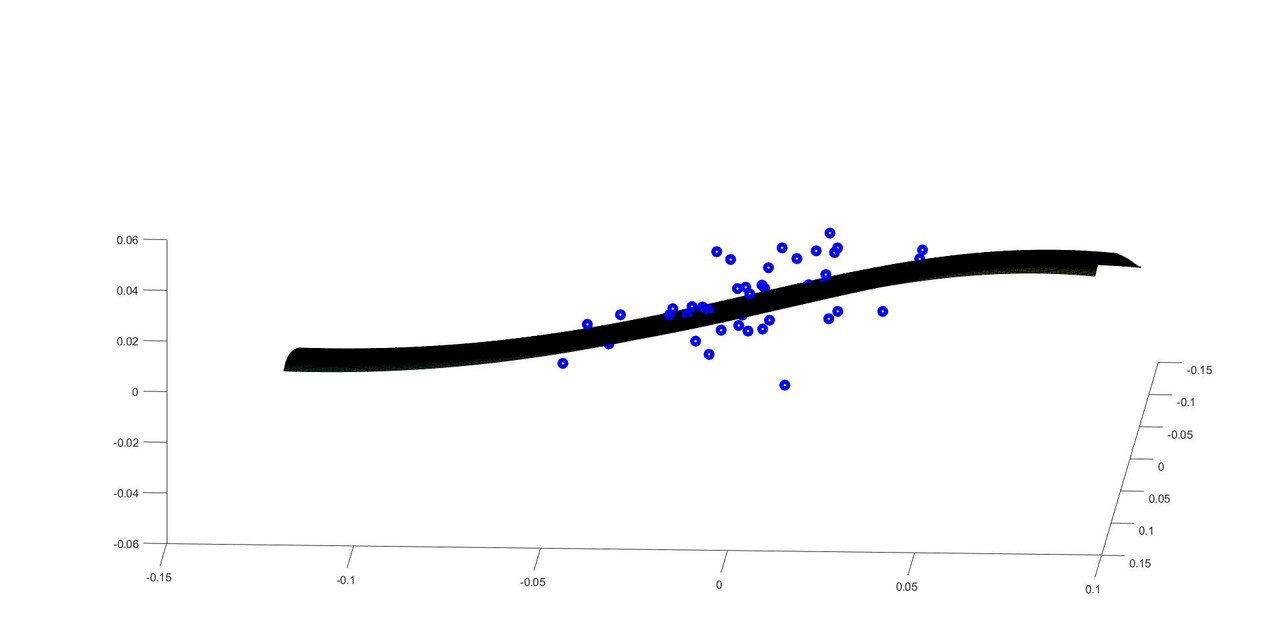Here are the regression analytics you will see.
First hermite correlations
CorrH(:,:,1) =

1.000000000000000   0.706373941869382   0.742423519593246
0.706373941869382   1.000000000000000   0.781142661662286
0.742423519593246   0.781142661662286   1.000000000000000

Second hermite correlations
CorrH(:,:,2) =

1.000000000000000   0.399838064591523   0.572052566256445
0.399838064591523   1.000000000000000   0.622166373120039
0.572052566256445   0.622166373120039   1.000000000000000

Third hermite correlations.
CorrH(:,:,3) =

1.000000000000000   0.338623911017974   0.481219060244469
0.338623911017974   1.000000000000000   0.542437187825566
0.481219060244469   0.542437187825566   1.000000000000000

Look at CorrH
Look at 2-D Graph

TSS =

0.017116089682868

0.005497822501914

ESS =

0.011777652804542

Rsquared =

0.678792142143499

Look at Regression numbers
You think life is a secret, Life is only love of flying, It has seen many ups and downs, But it likes travel more than the destination. Allama IqbalAmin
Topic Author
Posts: 2411
Joined: July 14th, 2002, 3:00 am

### Re: Breakthrough in the theory of stochastic differential equations and their simulation

Friends, I still have flu and light fever and hope to restart the work as soon as I get better.
I wanted to make more changes to the program but I knew that I would not be able to work further due to flu and decided to post the program.
For example densities constructed probably have to be based on sample variance, sample skew and sample kurtosis. I had been working with raw moments directly earlier. And I thought it was like an anomaly that sample central moments are biased and have to be adjusted while sample raw moments are unbiased. In next version of my program, I will make densities based on sample central moments after making required adjustments. There are further changes to graphing and some other parts of the program that I want to make. It is not a lot of work so new program should be ready one or two days after I get better.
I will try to find interesting applications of the hermite regressions. And then get back to resume the work on option pricing where I left to start working on regression models. I hope I will continue interesting work that would be helpful in research of many friends.
You think life is a secret, Life is only love of flying, It has seen many ups and downs, But it likes travel more than the destination. Allama IqbalAmin
Topic Author
Posts: 2411
Joined: July 14th, 2002, 3:00 am

### Re: Breakthrough in the theory of stochastic differential equations and their simulation

Friends, I was playing around with the new regression program and I noticed that using sample central moments without regard to raw moments deteriorates the fit while only increasing the fourth raw moment has a much better fit to data and much larger R^2. I might be doing something wrong and will double check everything again but it seems that using only central sample moments does not seem to work as well.
If all the densities involved are nice with good tails, the results are excellent but if some density had bad tails, it can deteriorate the results. Here is a very beautiful graph that I found when I was playing around.

StartI=01+5*46;%choose the start of the regression
EndI=46+5*46;%choose the last entry of the regression

The R^2 was .82, but I had slightly increased fourth raw moment further.
Here is the same graph with different angles.

.
.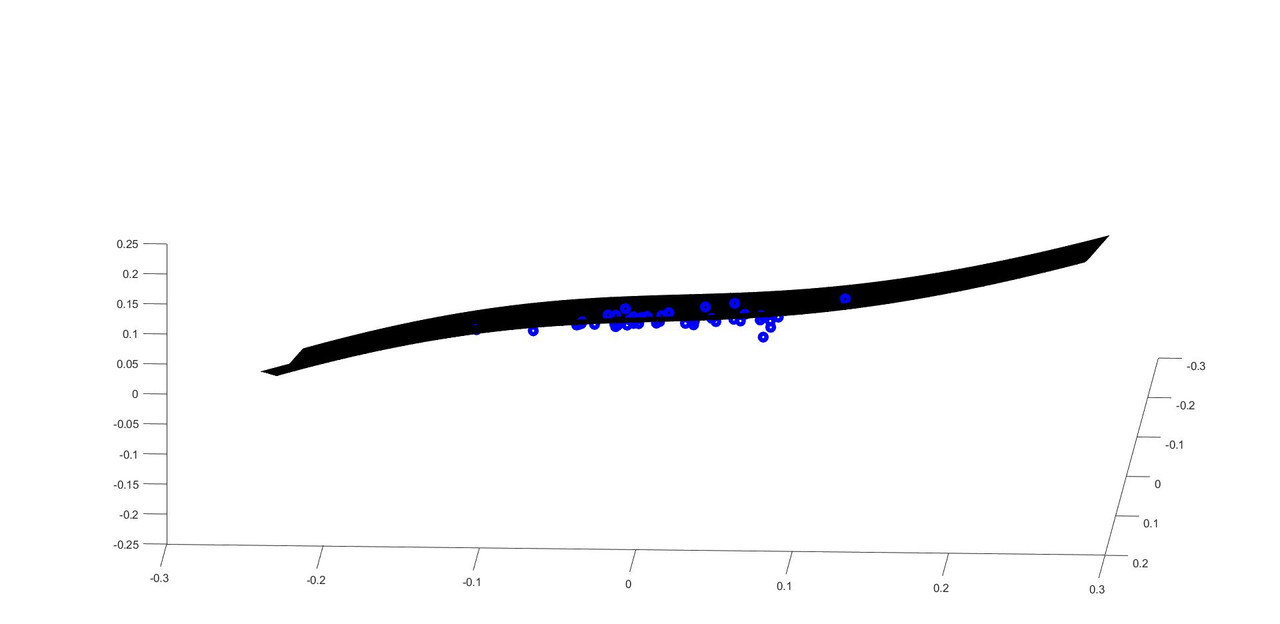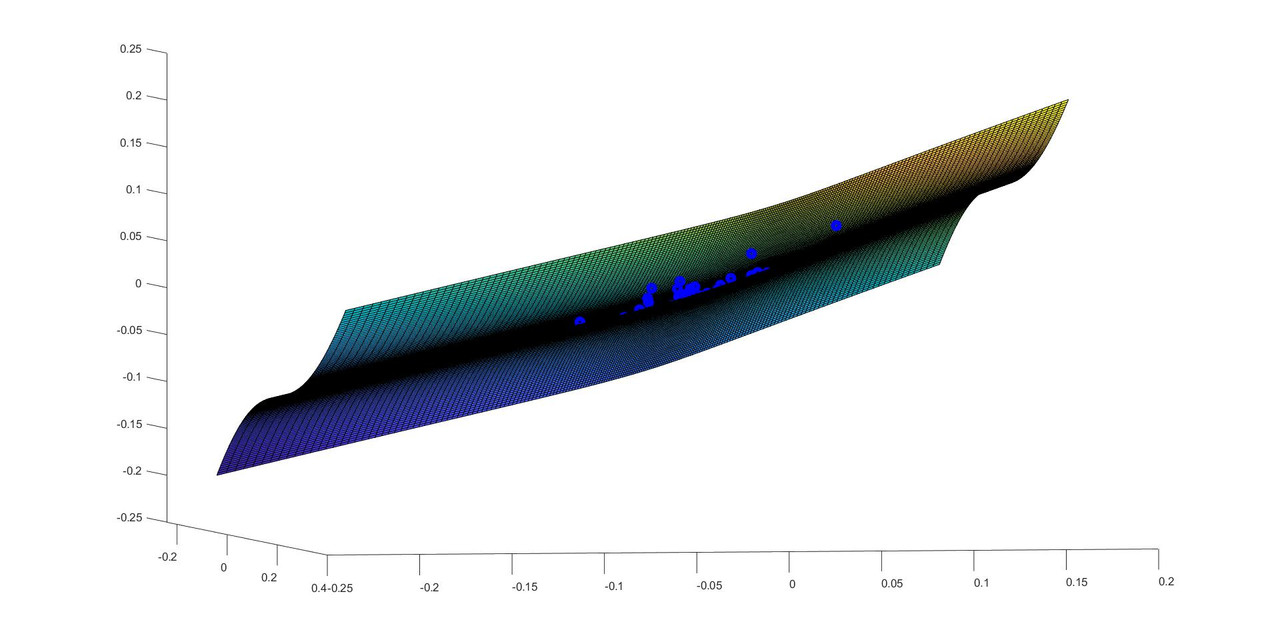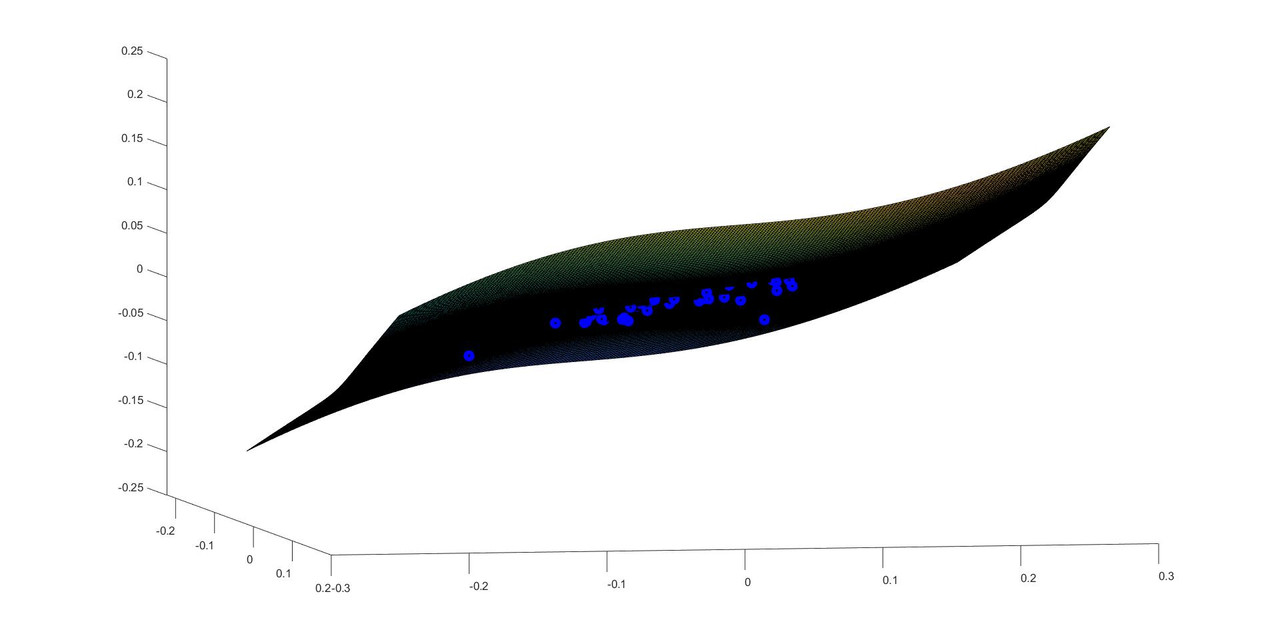You think life is a secret, Life is only love of flying, It has seen many ups and downs, But it likes travel more than the destination. Allama IqbalAmin
Topic Author
Posts: 2411
Joined: July 14th, 2002, 3:00 am

### Re: Breakthrough in the theory of stochastic differential equations and their simulation

It seems that problem is not whether we have sample moments and not population moments. The problem is that in many samples kutosis is too small for the density to have a positive first derivative (of X wrt Z as in Z-series) everywhere and it folds onto itself and larger Z's after a certain value of Z start to map on smaller values of the variable. This destroys the regression. It only requires that kurtosis is increased while keeping other moments constant. I will play and try to come with a new version of the program that increases kurtosis of the density until first derivative of the Z-series random variable with respect to Z is positive everywhere. In 90% of the densities, it will be a very small increase in value of kurtosis. I am sure it will fix all the problems.
In the meanwhile, please enjoy these two different graphs.
The first graph has R^2 .717 and second graph has R^2 .96
.
.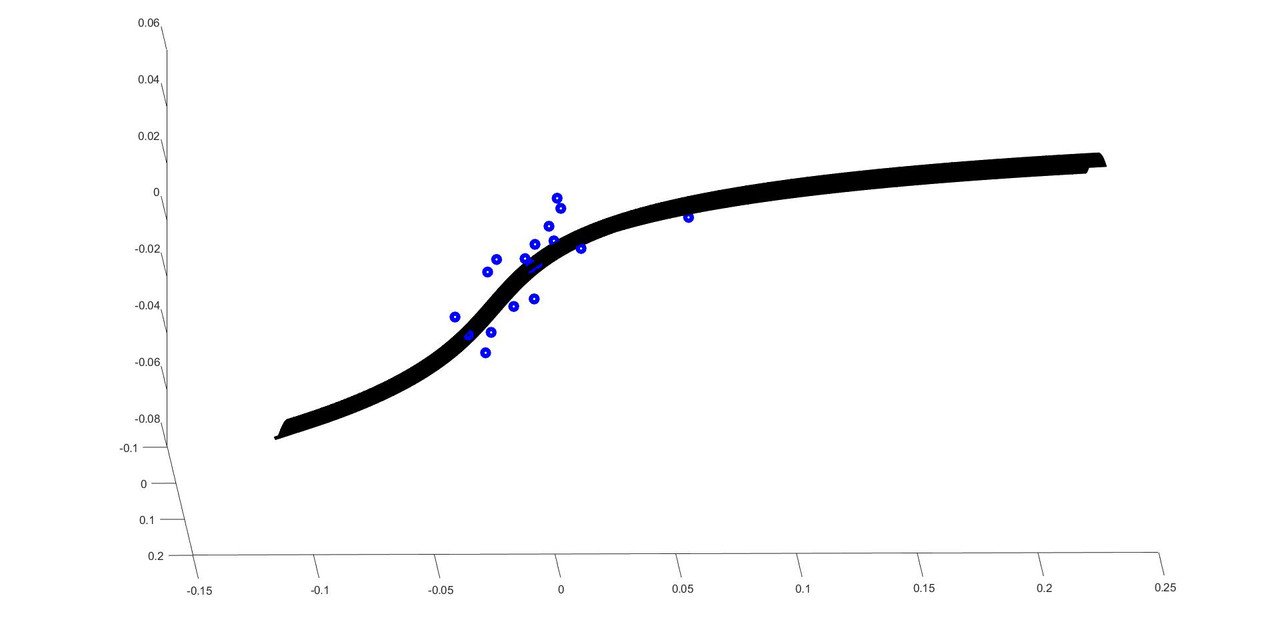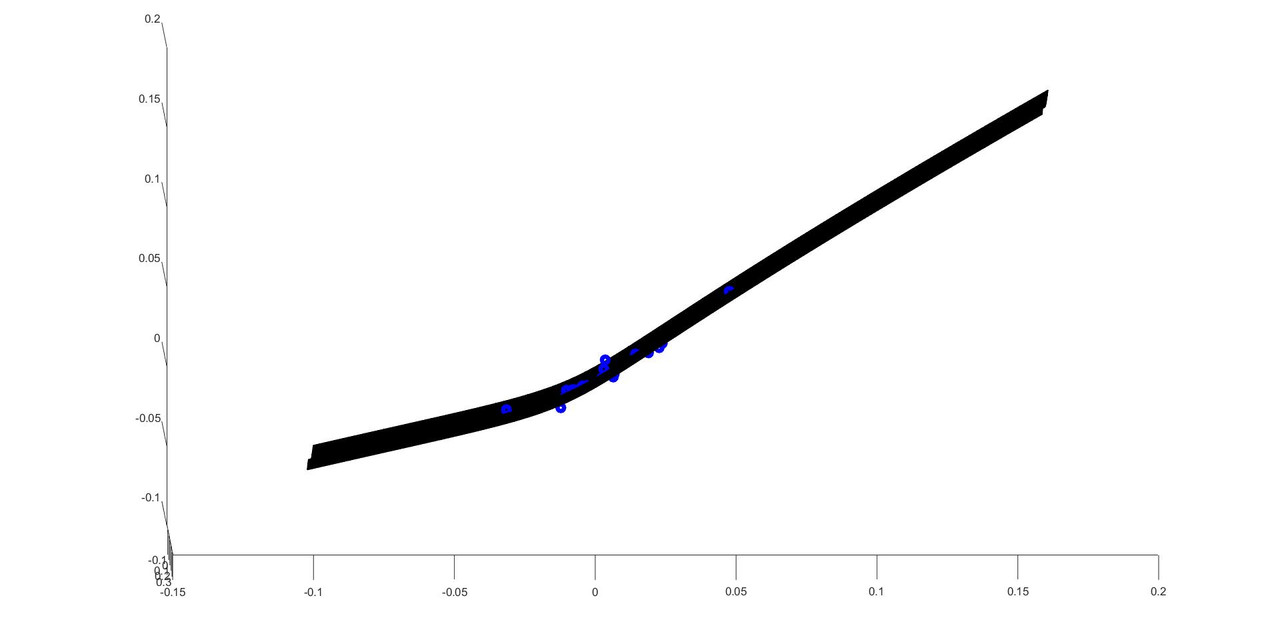You think life is a secret, Life is only love of flying, It has seen many ups and downs, But it likes travel more than the destination. Allama IqbalAmin
Topic Author
Posts: 2411
Joined: July 14th, 2002, 3:00 am

### Re: Breakthrough in the theory of stochastic differential equations and their simulation

Friends, while finding marginal densities for our hermite regression framework, we have tried to fit four moments. That means that valid equation for Z-series random variable underlying density would be

$X\, = \, a_0 \, + \, a_1 \, Z \, +\, a_2 \, Z^2 \, +\, a_3 \, Z^3 \,$

The first derivative of X with respect to Z is given as

$\frac{dX}{dZ}\, = \, a_1 \, +\, 2\, a_2 \, Z \, +\, 3\, a_3 \, Z^2 \,$

In order to have a nicely behaved density, we need  $\frac{dX}{dZ}\,>0 \,$  everywhere. So as Z increases, X also increases. If somewhere   $\frac{dX}{dZ}\,<0 \,$ , the value of X would start to decrease when Z increases and we see the tail of the density folding backwards onto itself as a result.
Luckily for four moments fit, our $\frac{dX}{dZ}\,$ is only a quadratic variable and we know the condition that in order to always remain positive our quadratic polynomial does not have any real roots (We can  probably let them have negative root beyond 5 or six standardized devs from median on both sides. Just possibly) .

So our condition for a nice density is that     $\frac{dX}{dZ}\, = \, a_1 \, +\, 2\, a_2 \, Z \, +\, 3\, a_3 \, Z^2 \,= \,0$   does not have a real root.

which requires from quadratic formula that  $b^2 \, - \, 4 \,a \, c < 0$    where $a=3 \, a_3$ , $b\, = 2\, a_2$ and $c \,= \, a_1$
and
$b^2 \, - \, 4 \,a \, c = (2 \, a_2)^2 \, - 12 \, a_3 \, a_1 \, <0$
$= \, {a_2}^2 \, - \, 3 \, a_3 \, a_1 <0$

$a_2$ is coefficient required for mostly skew while $a_1$ and $a_3$ are for large part related to variance and kurtosis respectively.
Too large skew with smaller variance or kurtosis causes density to fold back on itself by causing  $\frac{dX}{dZ}\,<0$ somewhere in the tails.
So one way could be to slightly change the density to increase kurtosis/fourth moment while possibly decreasing skew a slight bit  and keeping second moments and variance constant until the condition for a nice density is properly fulfilled. For most densities this correction would be very little but once corrected, regression results would be perfectly nice and we would have always good and well behaved regressions.
I will work with this and possibly come back in a day or two with a better program where our multivariate regression always works.
You think life is a secret, Life is only love of flying, It has seen many ups and downs, But it likes travel more than the destination. Allama IqbalAmin
Topic Author
Posts: 2411
Joined: July 14th, 2002, 3:00 am

### Re: Breakthrough in the theory of stochastic differential equations and their simulation

Friends, I want to mention that an antipsychotic injection is due on coming Monday so I may not be able to work very well after a few days. I have requested psychiatrist's office to arrange a phone meeting which should possibly follow in next few days. I really want to ask the doctor to end my injections and antipsychotic medication as I am perfectly mentally healthy. Many psychiatrists in Pakistan force antipsychotics on healthy people and also detain them for long periods of time under pressure and coercion from Pakistan army (whose generals receive tens of millions of dollars in bribes from US army to facilitate mind control of intelligent people in Pakistan).
I also want to request European embassies in Pakistan to keep a vigil and record my phone call with the psychiatrist so they would know the tactics of CIA, Pakistan army and psychiatrists to gain an understanding of mind control abuse since a lot of very talented Europeans suffer from a very similar abuse on hands of corrupt generals in American army(whose many generals are desperate to do anything to please their billionaire Goldfather and would gladly and obediently retard muslims, blacks, Europeans or even Americans who are talented  when Goldfather wishes them to do so).
I also want to request American and Pakistani friends if they can possibly approach my psychiatrist  (Far*** A. Minhas of treehouse, Peshawar road, Rawalpindi) to tell him about horrors of mind control abuse and stop following the orders of Pakistan military. Many times these psychiatrists are not bad people but they are told concocted lies and false ethical justification about mind control by generals who would have already taken large sums of bribes. I am sure many of these psychiatrists would be very careful about mistreating  their patients once they know the truth. But I cannot take this risk on my own since there is a huge possibility that doctor might even increase my medication several fold if I say this is all a conspiracy. It is unbelievably moronic but I have read it myself in psychiatry books that whoever claims that secret services are after me is schizophrenic.
You think life is a secret, Life is only love of flying, It has seen many ups and downs, But it likes travel more than the destination. Allama IqbalAmin
Topic Author
Posts: 2411
Joined: July 14th, 2002, 3:00 am

### Re: Breakthrough in the theory of stochastic differential equations and their simulation

Friends, I wrote a small function that increases fourth moment in calibration until our positivity of first derivative dX/dZ condition is satisfied. Now regression seems to give very good results. I quickly went ahead and divided the data I had into 27 blocks of ten days each. As daily returns were calculated, there were only nine entries in each regression for each block of ten days. I plotted the 2-D graphs and tried to get the best view after rotating each graph. Here are 27 graphs which have APPL returns VS MSFT and NVDA returns for ten days each(nine returns each).
I will post this new program in a few hours that I used to generate these graphs.
b11 and b21 are standardized regression coefficients for MSFT and NVDA for explaining first hermite deviations in APPL
b12 and b22 are standardized regression coefficients for MSFT and NVDA for explaining second hermite deviations in APPL
b13 and b23 are standardized regression coefficients for MSFT and NVDA for explaining third hermite deviations in APPL
vh1, vh2, and vh3 are volatility coefficients of APPL for first, second and third hermite respectively.
These coefficients are given on title of the graphs.
Here are the graphs.
.
.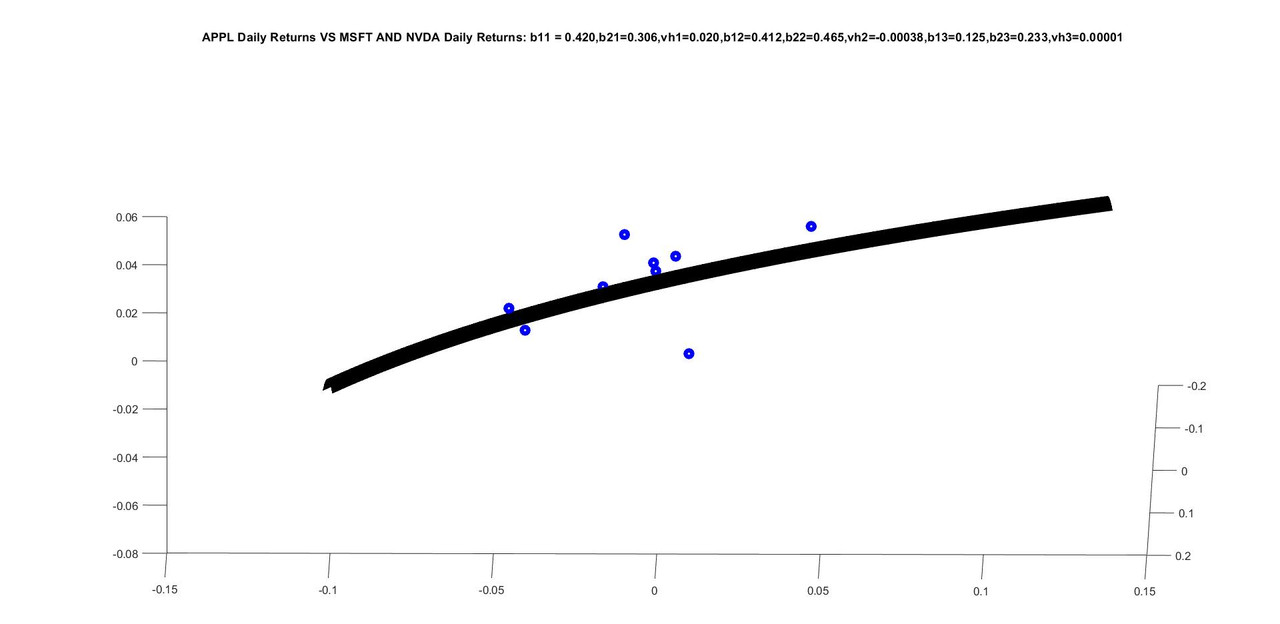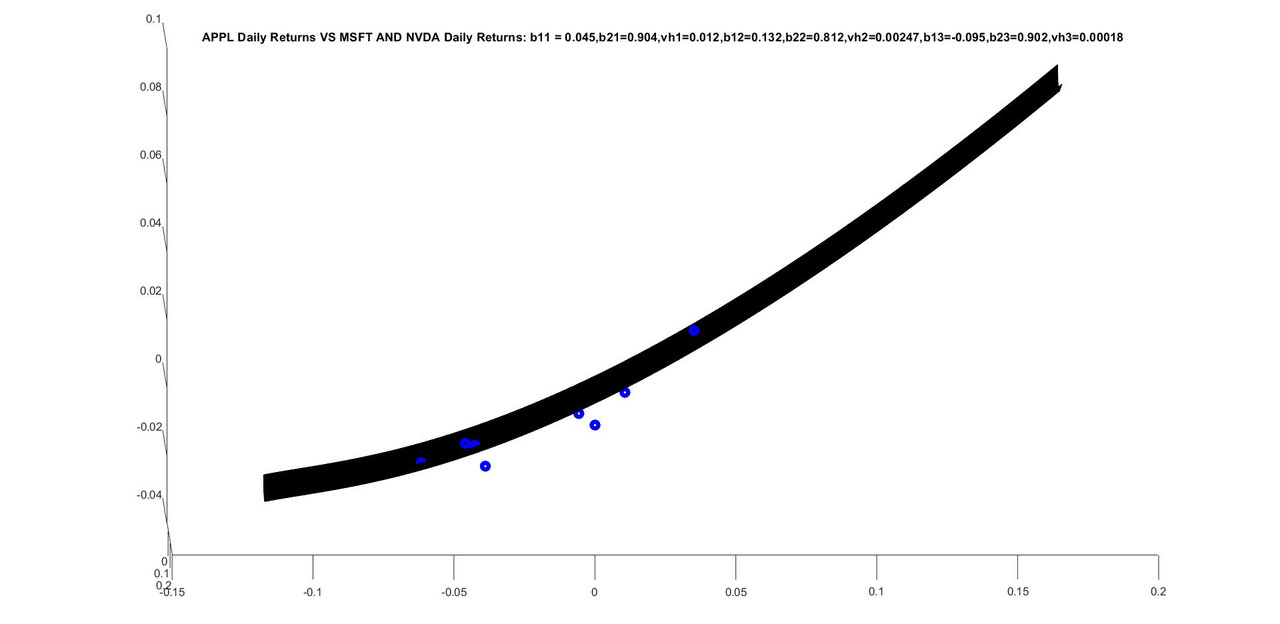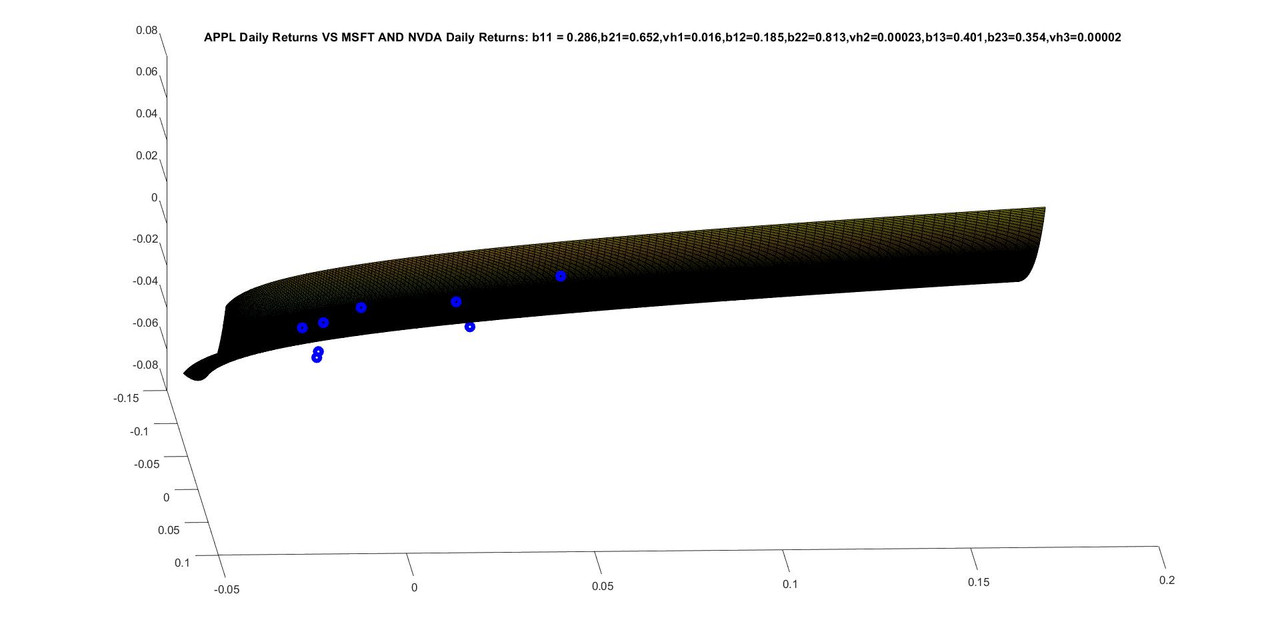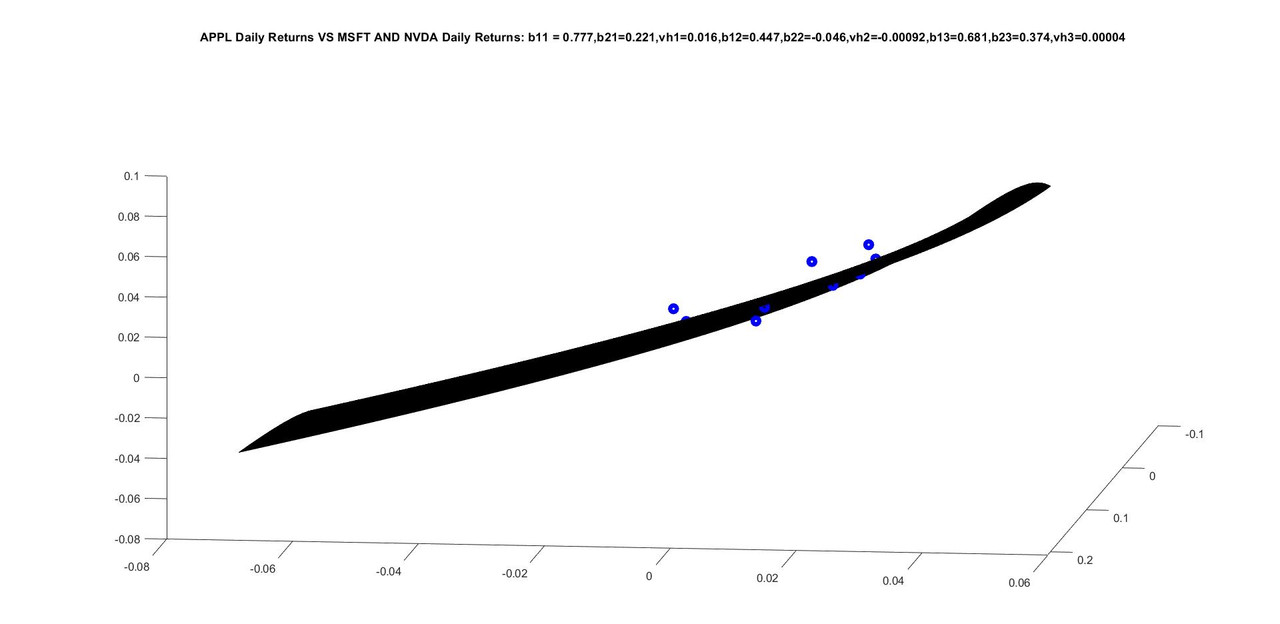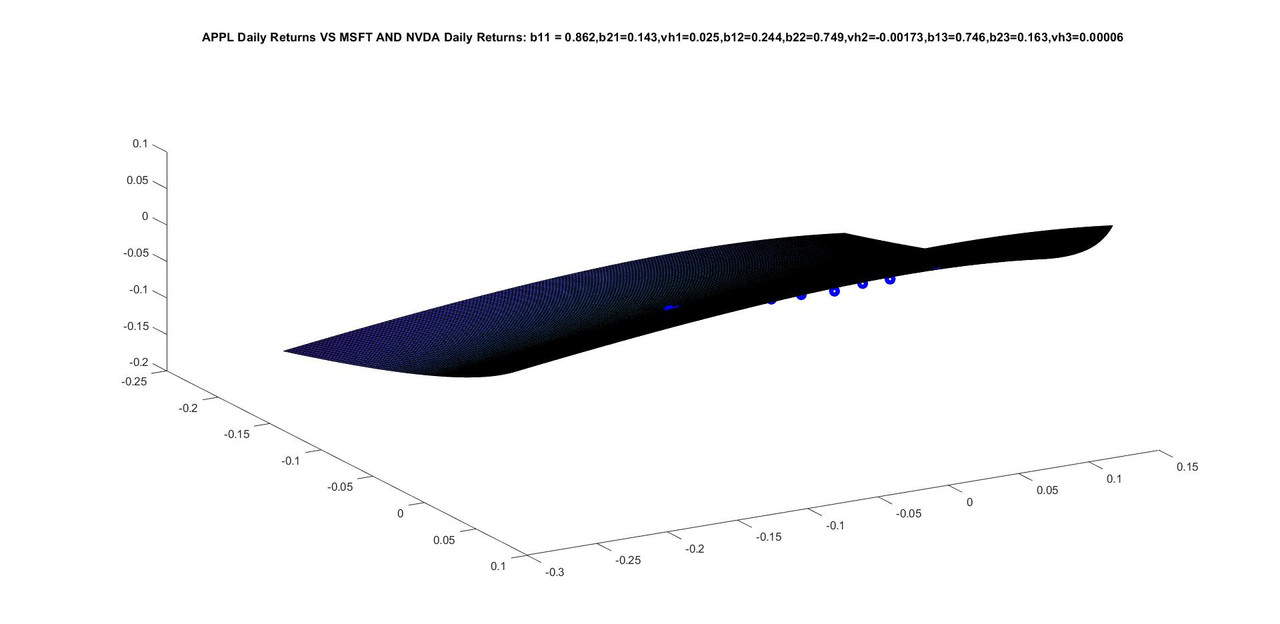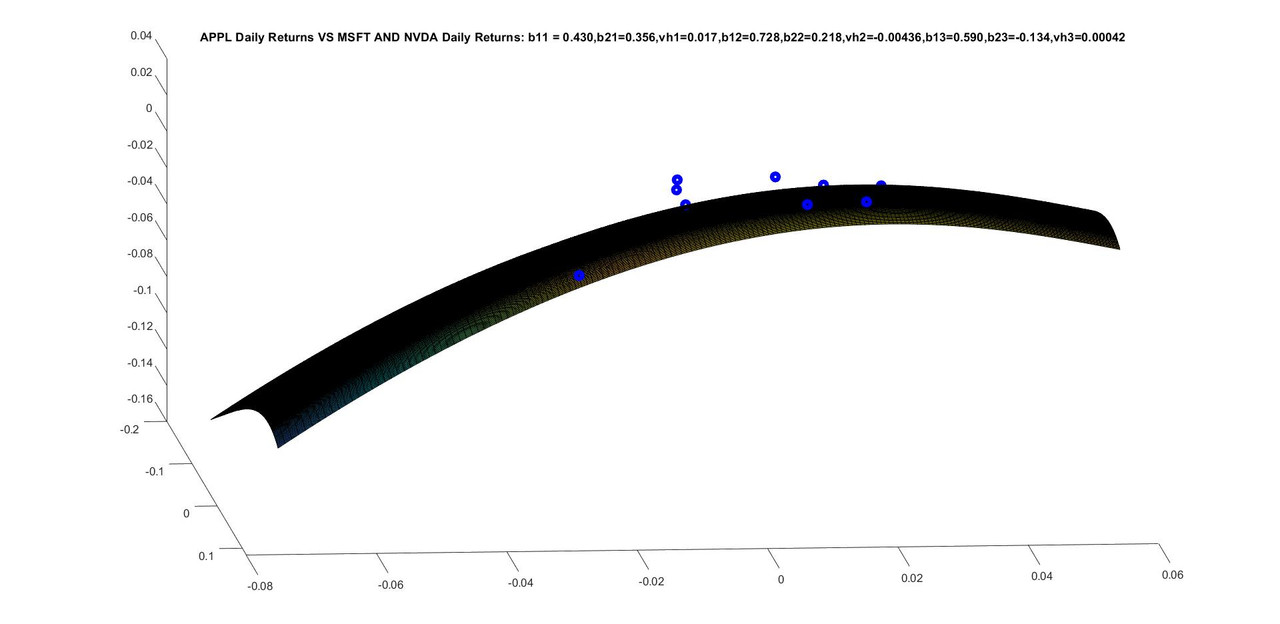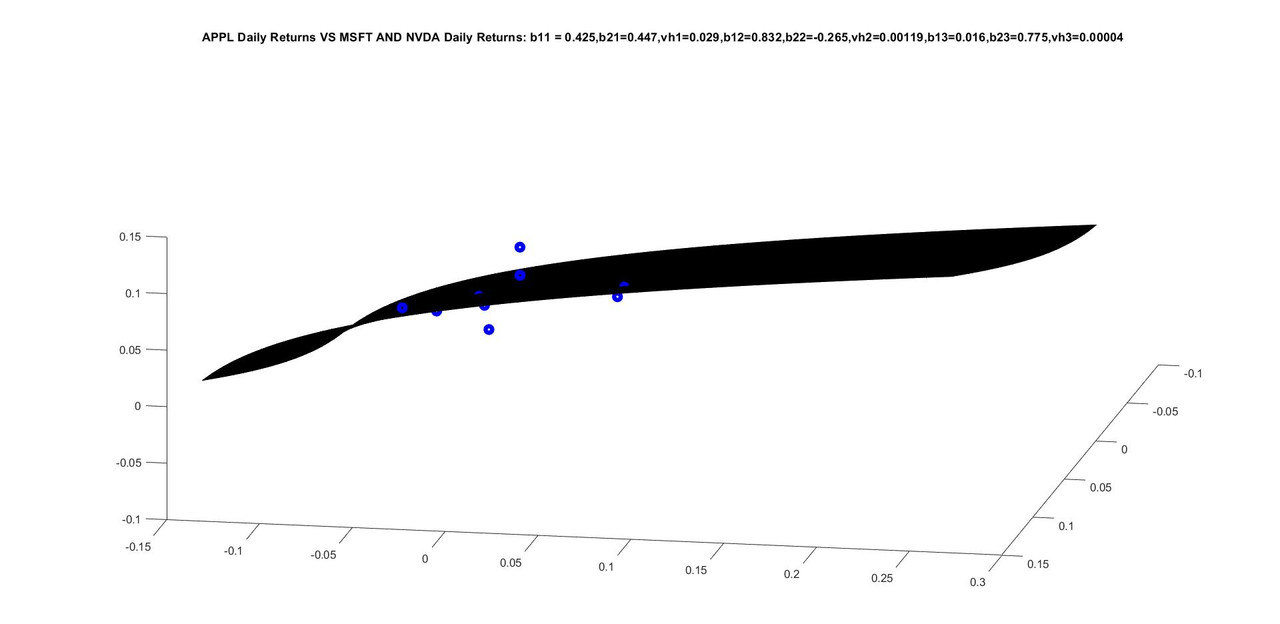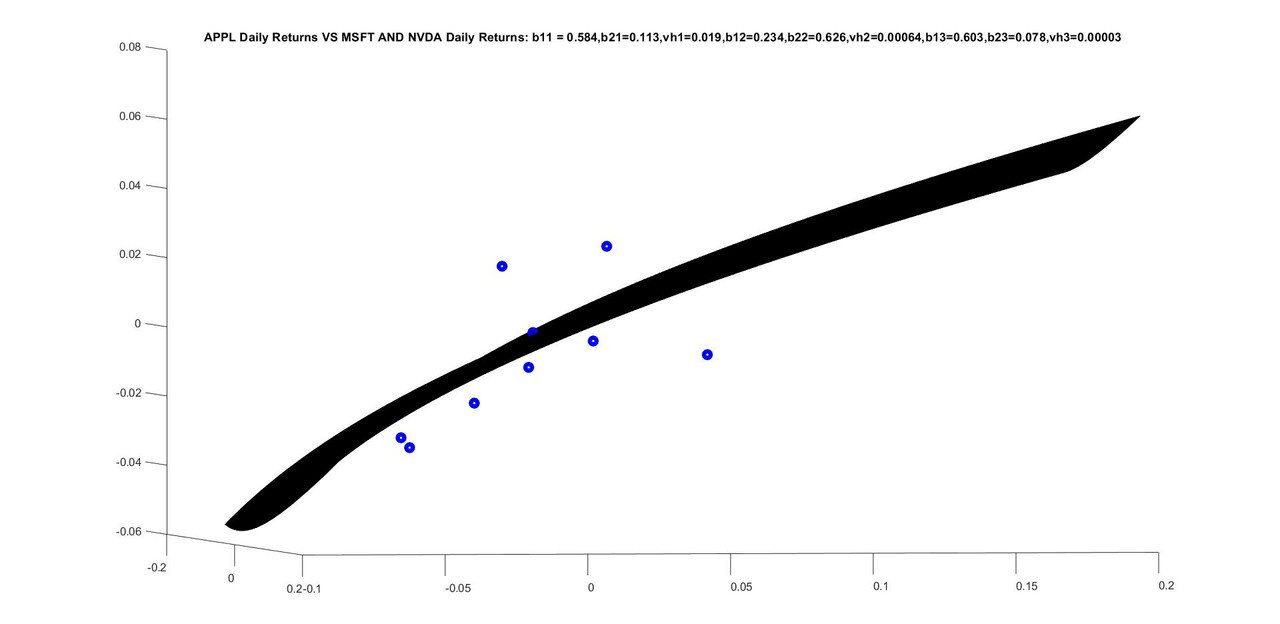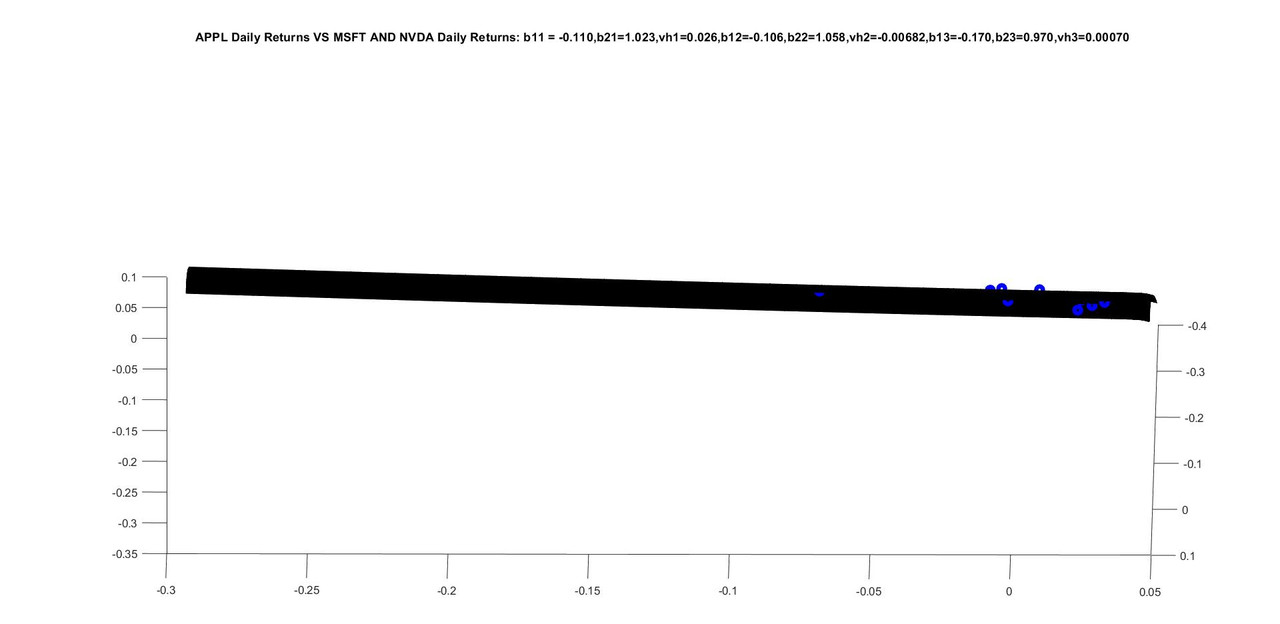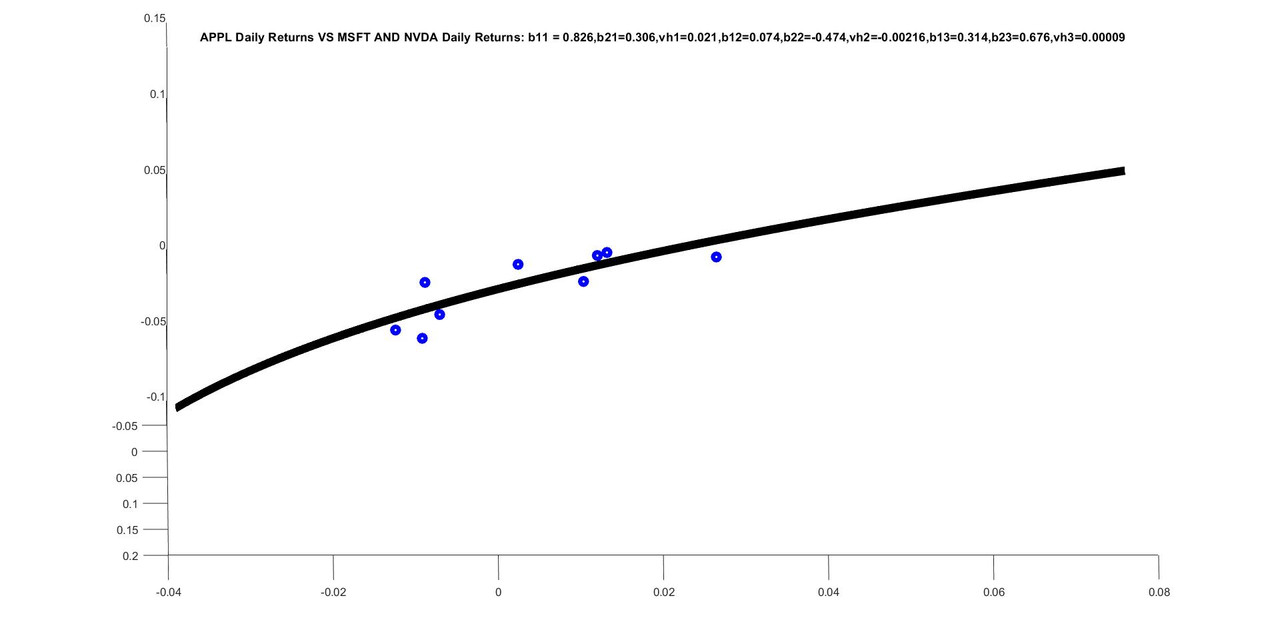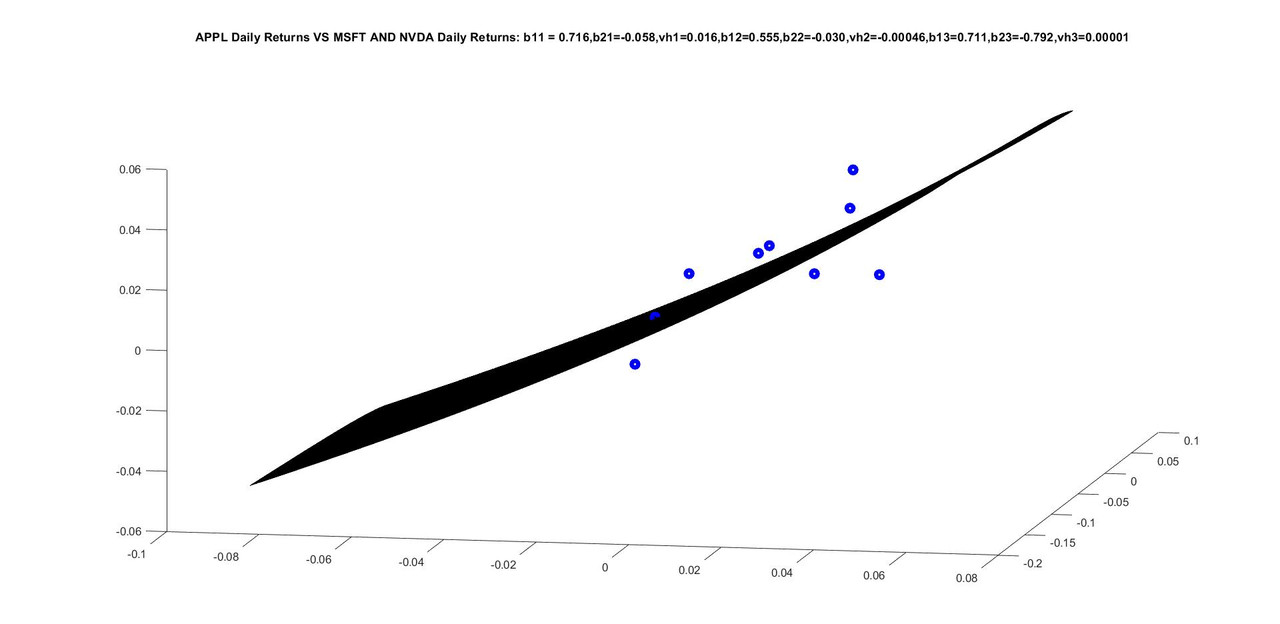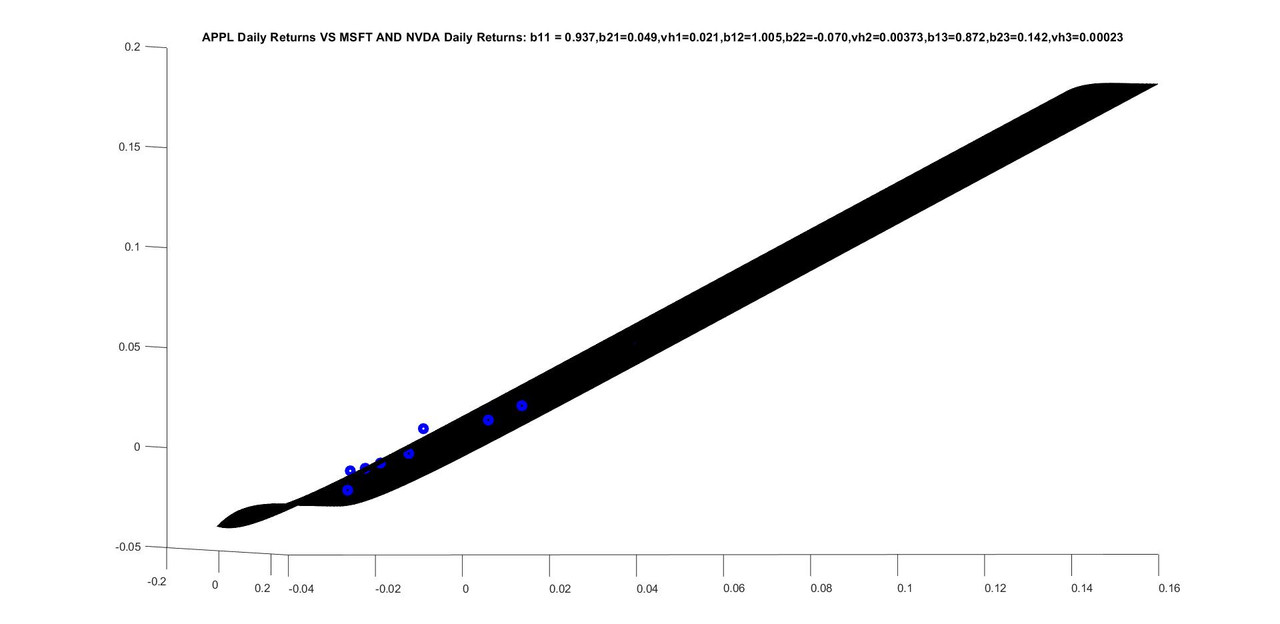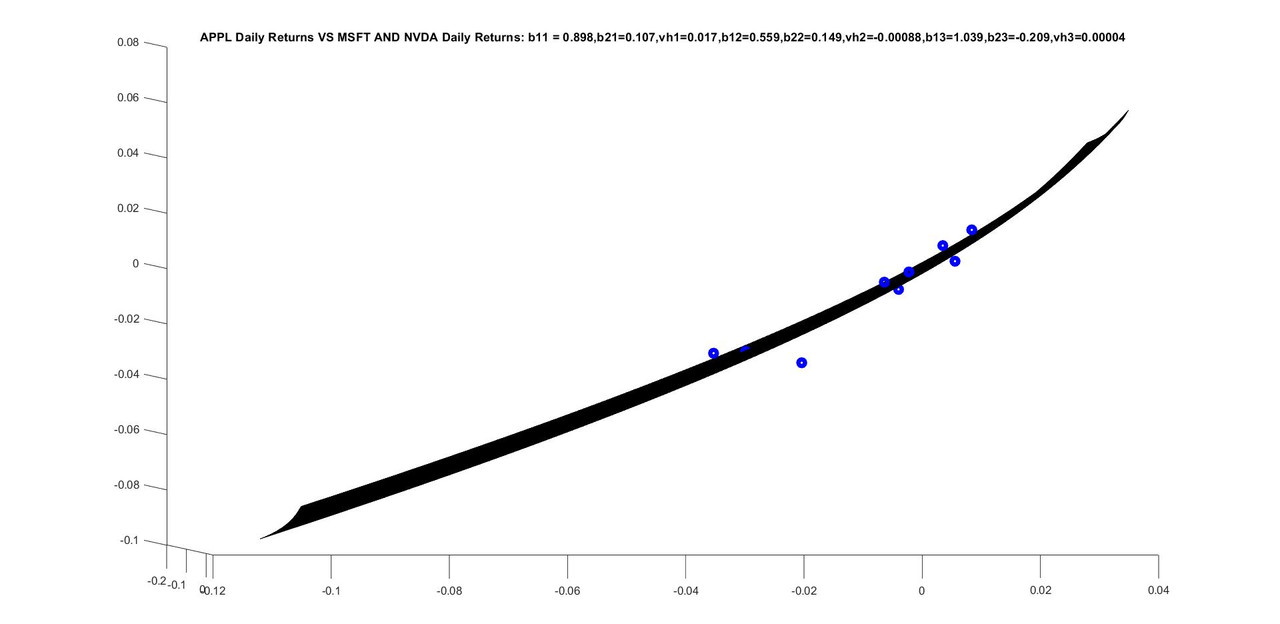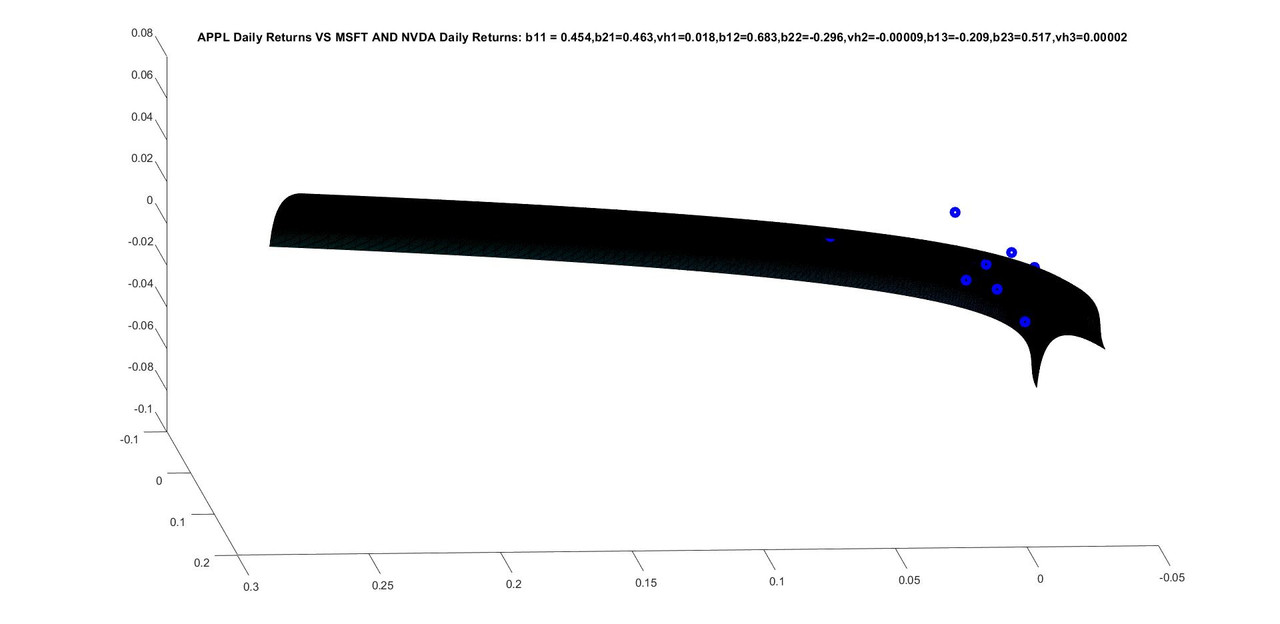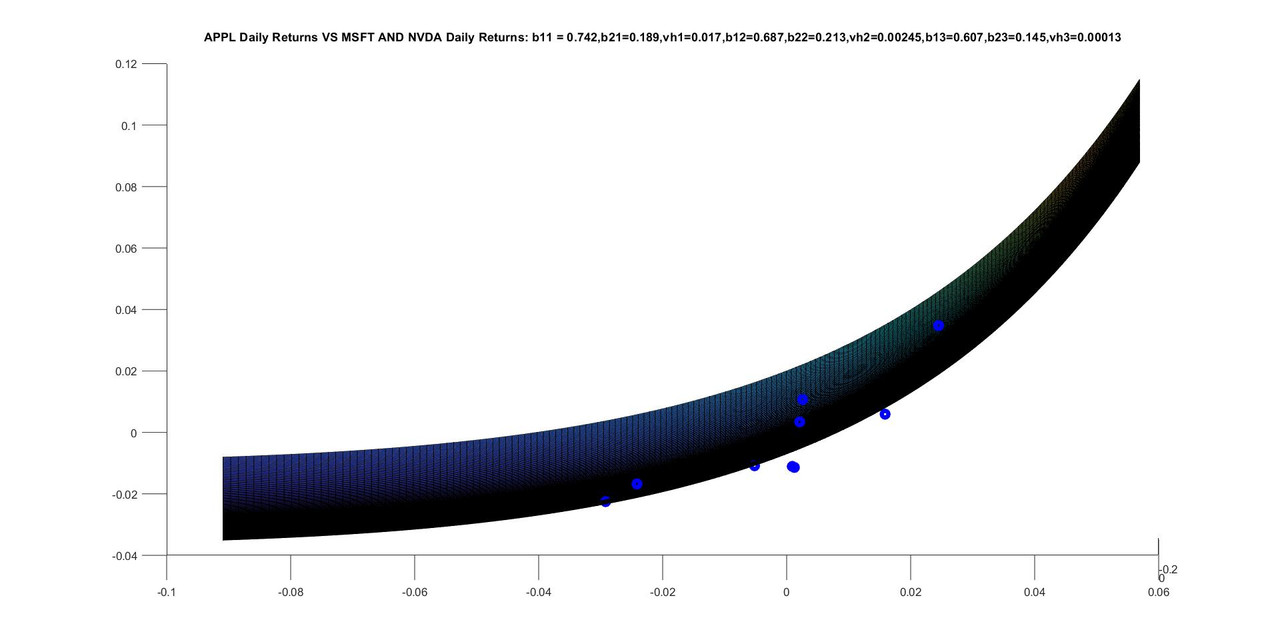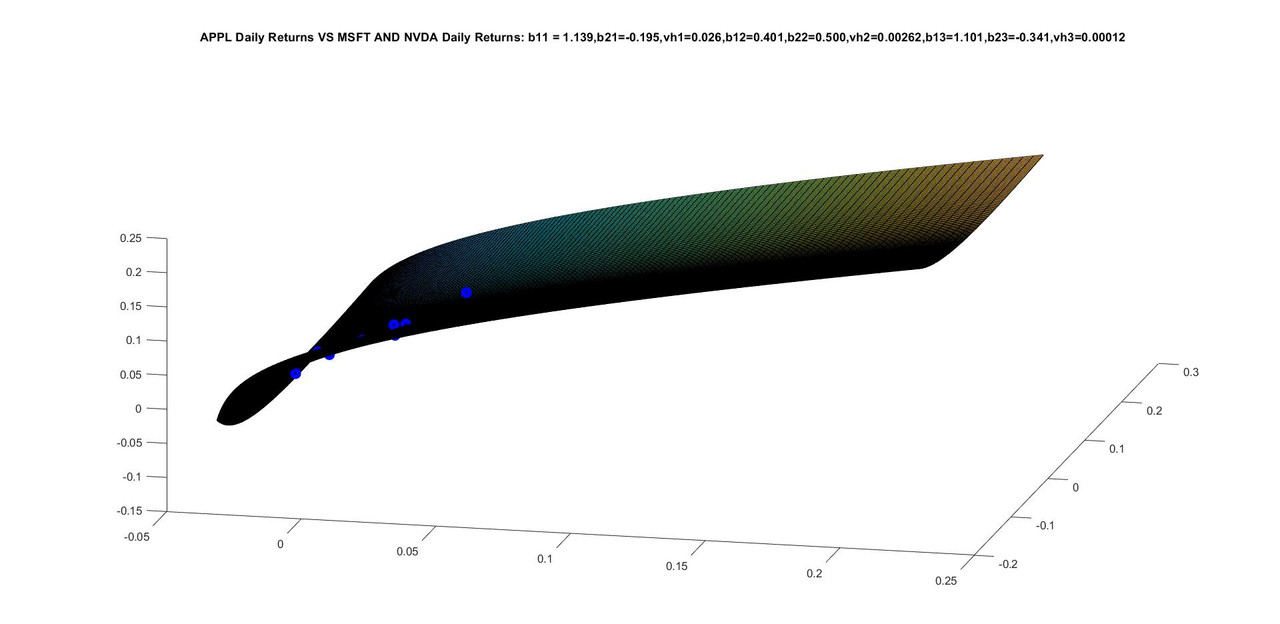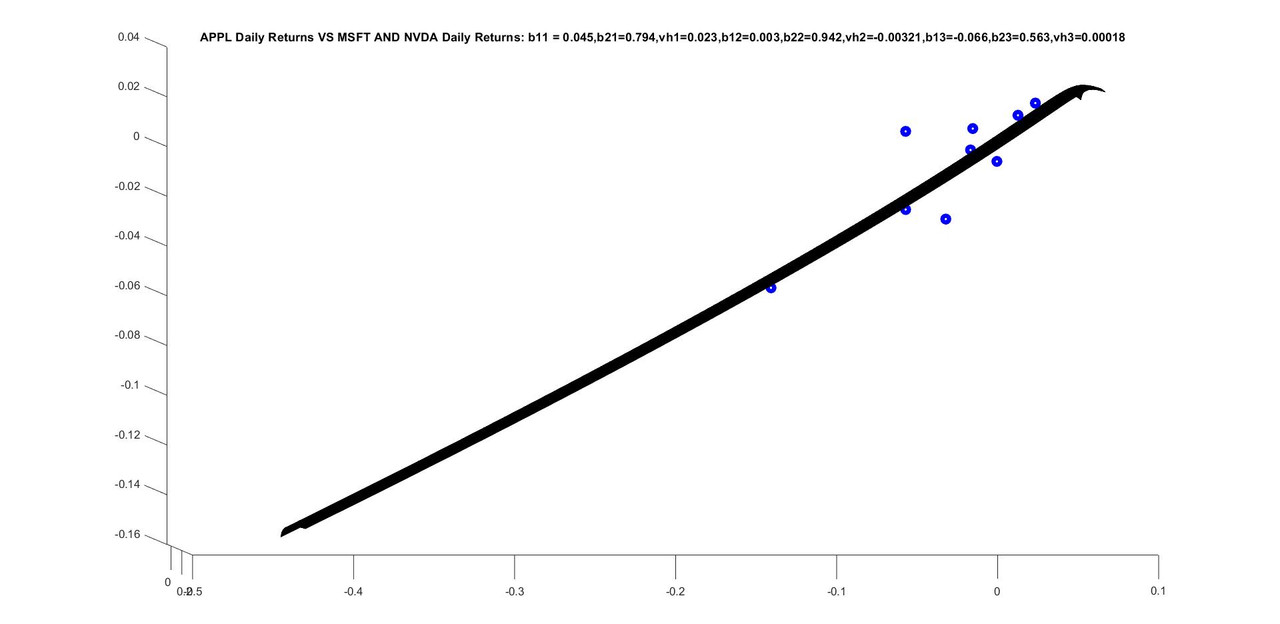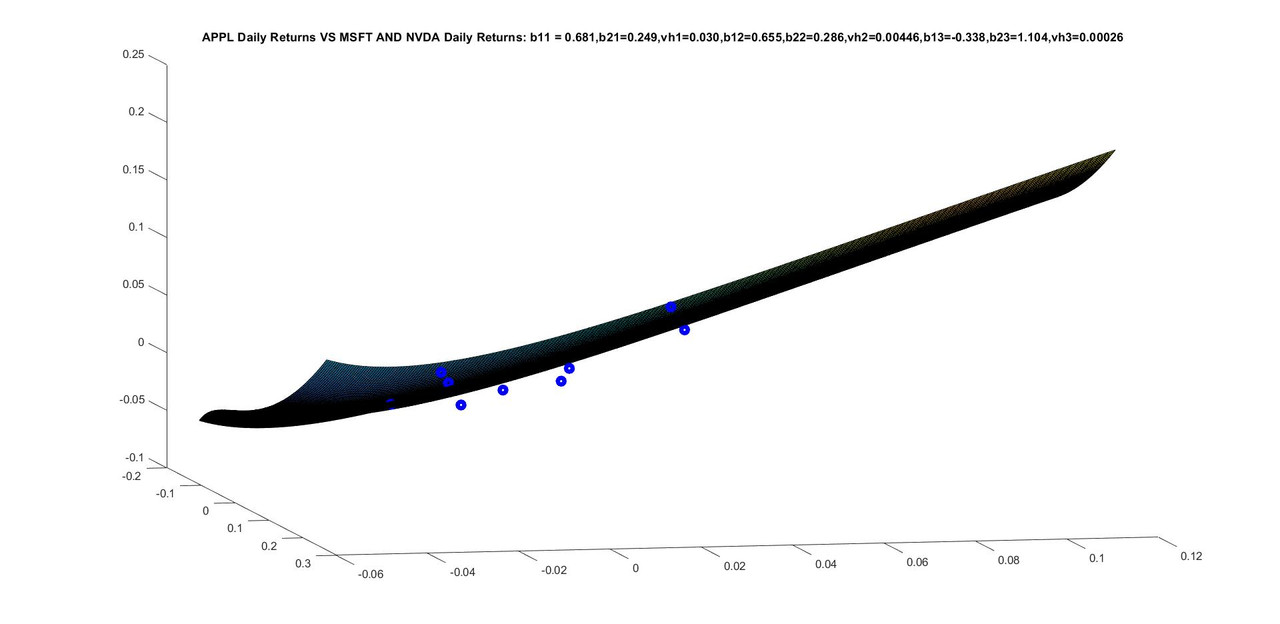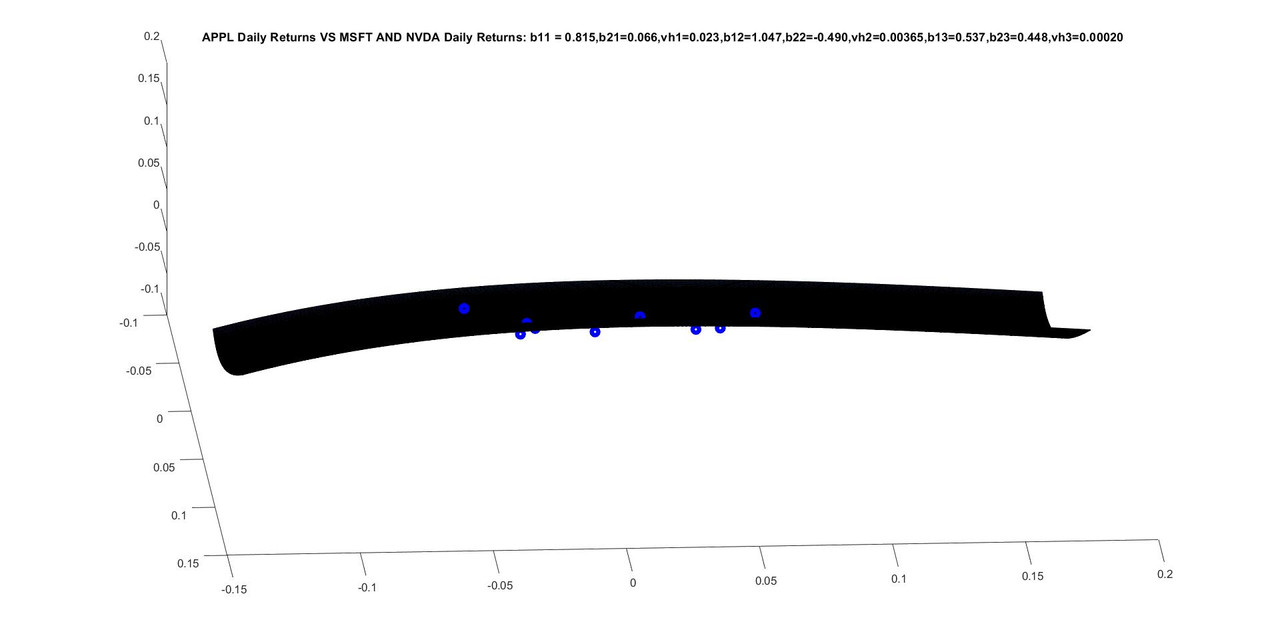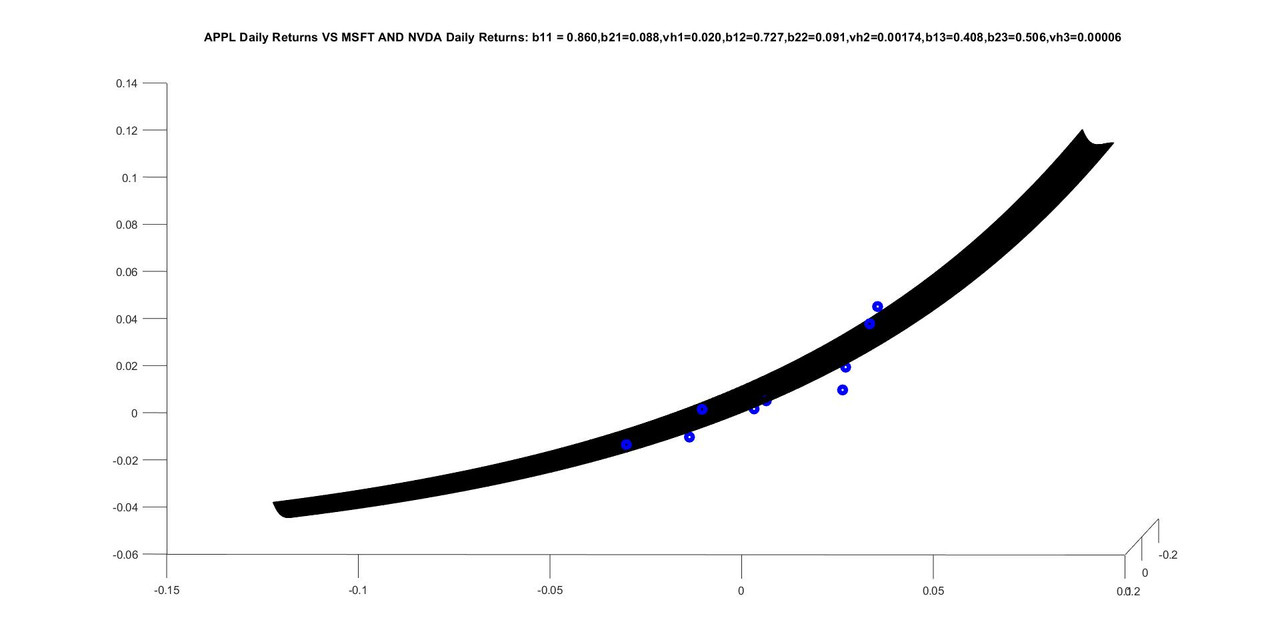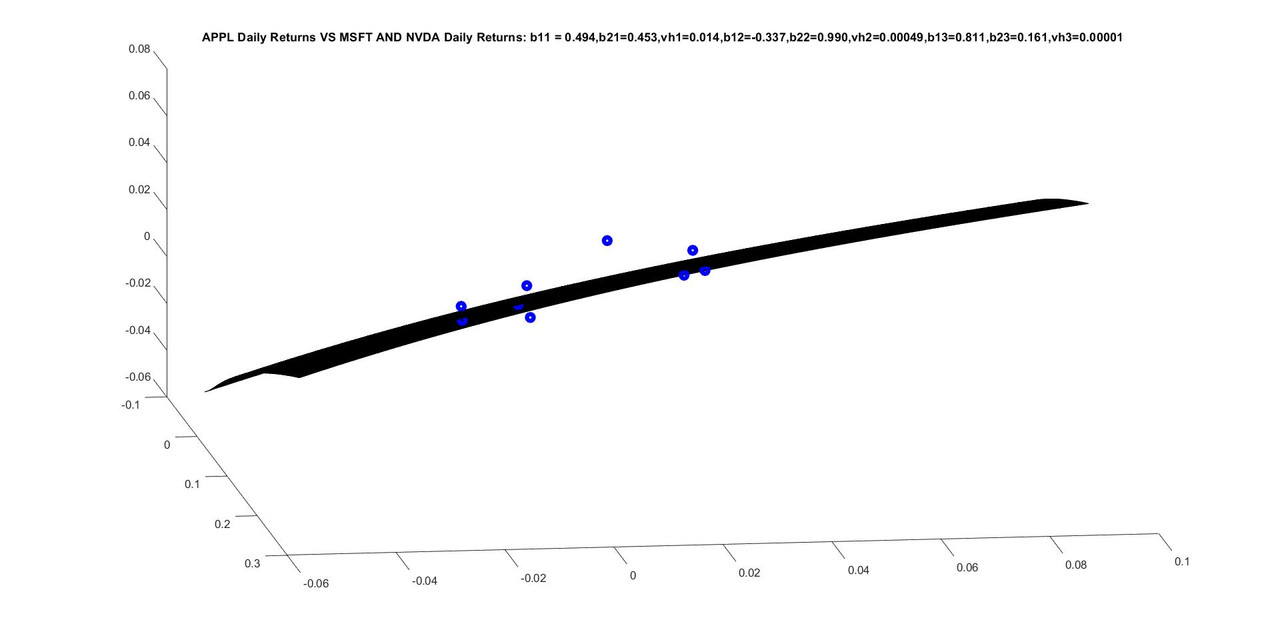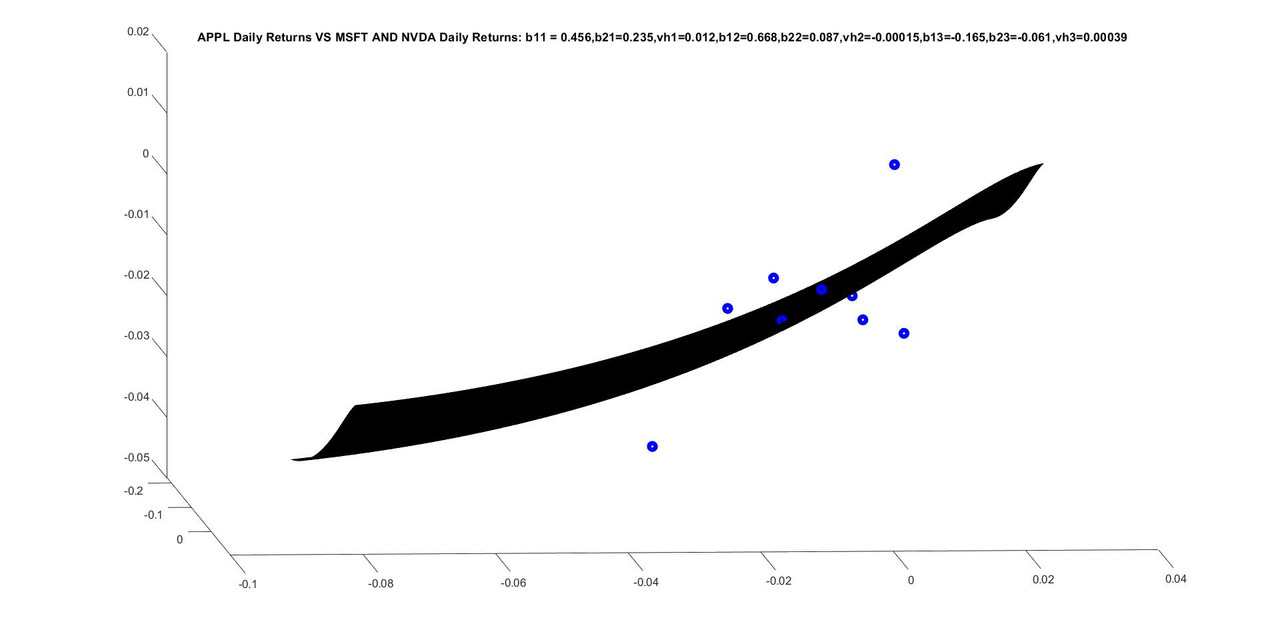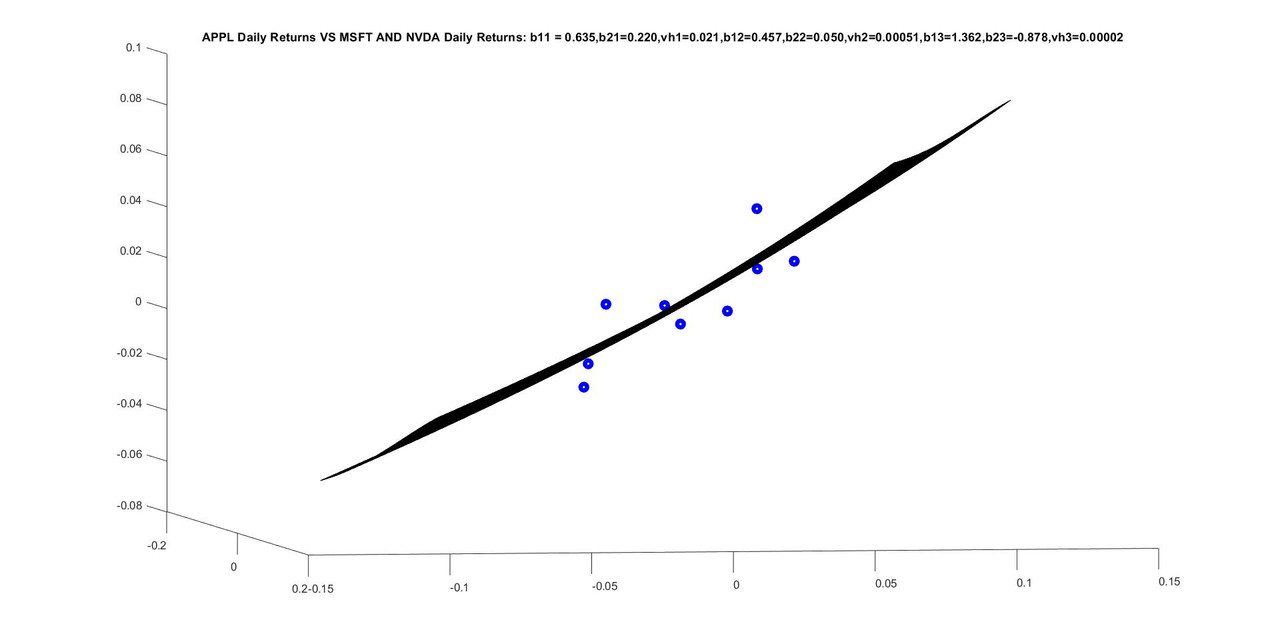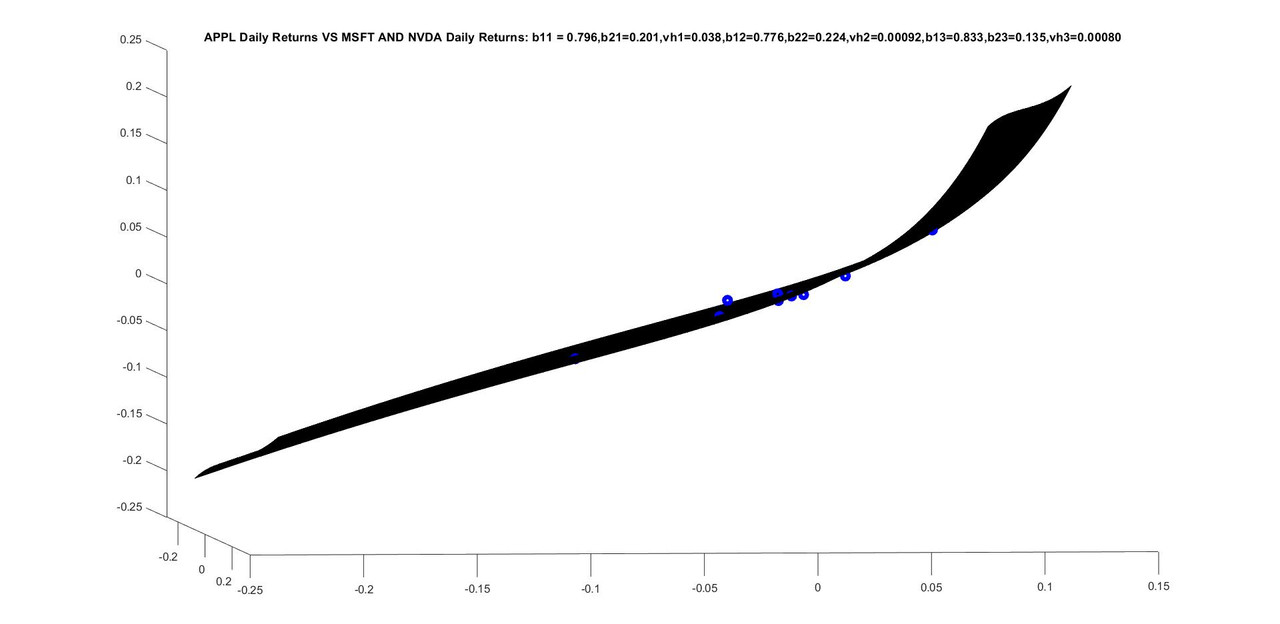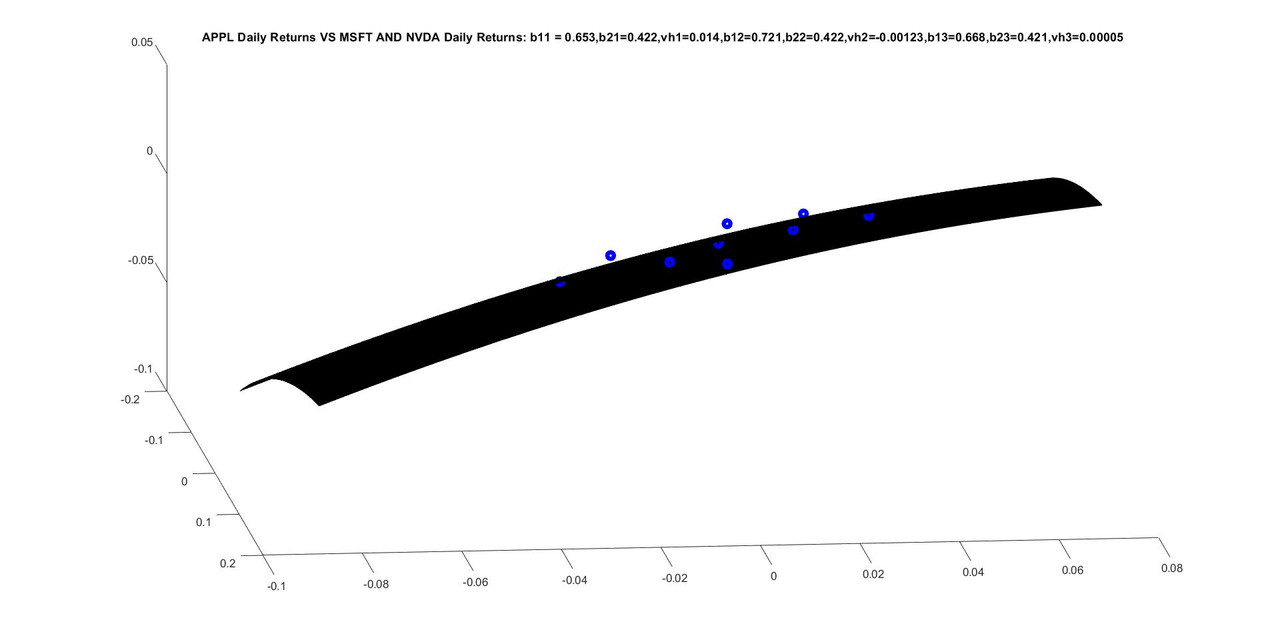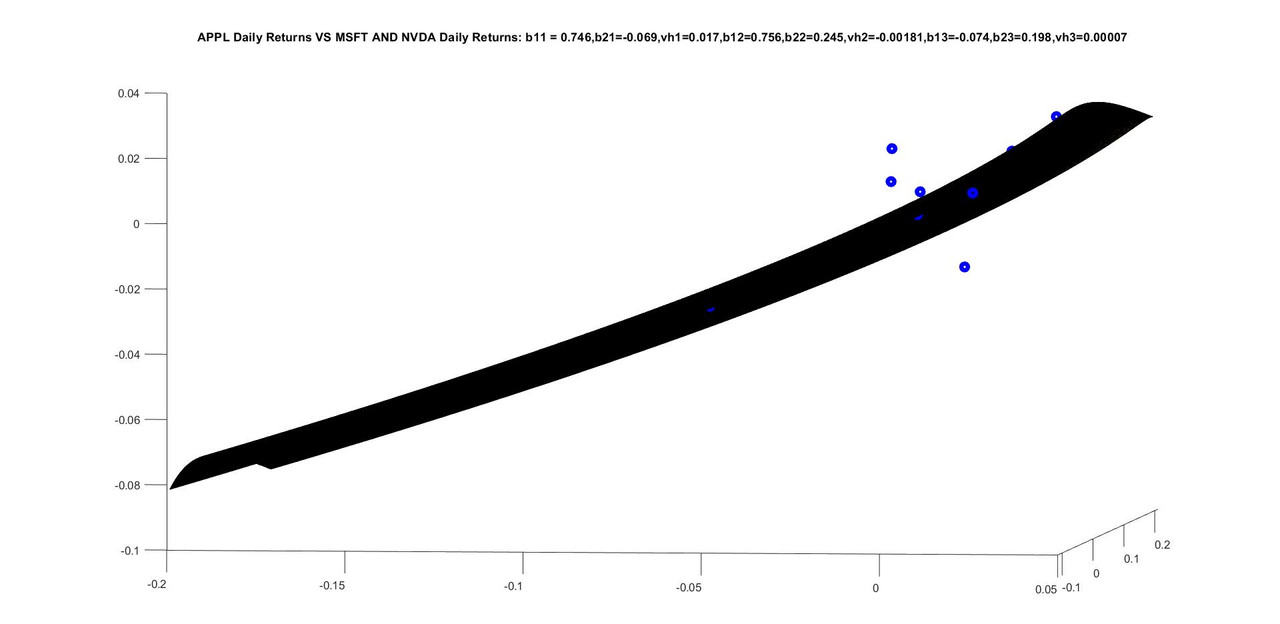You think life is a secret, Life is only love of flying, It has seen many ups and downs, But it likes travel more than the destination. Allama IqbalAmin
Topic Author
Posts: 2411
Joined: July 14th, 2002, 3:00 am

### Re: Breakthrough in the theory of stochastic differential equations and their simulation

Here are the updated files that are needed to make new changes to the program. Rest of the files are the same. I will post a new updated version in a few days but quickly posting the new program so that fourth raw moment is fixed at first derivative dX/dZ positivity condition as described in a previous post.
.
.
function []= DoMultivariableMultiHermiteRegression(Y1,X1,MM,TT,HH)

W(1:MM,1:TT)=X1(1:MM,1:TT); %We add regressors to W
W(MM+1,1:TT)=Y1(1,1:TT);% We add regressand to W
%Above we added all explanatory and explained variables in a single matrix
%so that we could calculate correlations between respective Z-values of the
%W matrix.

for mm=1:MM+1  %total number of variables is number of regressors + 1(which is regressand)
X(1:TT)=W(mm,1:TT); %Extract the time series of each variable in W
rMu(mm,:) = CalculateFourRawMomentsFromData(X,TT);%Calculate four raw moments of each time series.
%[c0(mm),c(mm,:)] = CalculateZSeriesDensityFromRawMomentsM4(rMu(mm,:));%Calculate Z-series from each moment

[c0(mm),c(mm,:)] = CalculateZSeriesDensityFromRawMomentsFix4thMoment(rMu(mm,:));
%[cMu(mm,:),Mu1(mm)] = CalculateFourCentralMomentsFromDataA(X,TT);%Calculate four raw moments of each time series.
%[c0(mm),c(mm,:)] = CalculateZSeriesDensityFromCentralMomentsM4(cMu(mm,:),Mu1(mm));%Calculate Z-series from each moment

PlotZSeriesDensity(c0(mm),c(mm,:),'g');%Look at the density to make sure it is valid. If the tails fall
%backwards in either direction, regression result can be flawed.
Z(mm,1:TT)=CalculateZgivenXAndZSeriesC3(X,c0(mm),c(mm,:));%Calculate Z for each data entry in time series of every variable(one by one through loop)
[ch0(mm),ch(mm,:)]=ConvertZCoeffsToHCoeffs(c0(mm),c(mm,:),3);%Convert the Z-coeffs to H-coeffs

end

%below we calculate regression coefficients between standardized Z-values
%of regressors and regressand. We only use correlations and covariances do
%not enter this calculation.

NN=MM+1; %This is regressors + 1 which is explained variable.
%Z
%str=input('Look at Z');
%Z is a matrix containing standardized Z's of both regressors and
%regressand and we calculate all cross correlations in it.Three different
%correlation matrices are calculated each for 1st, second and third hermite
%as we are using three hermites in our analysis.
CorrH = CalculateCorrelationMatricesHermiteCH(Z,NN,TT,HH)
str=input('Look at CorrH');
for hh=1:HH %Loop over all hermite polynomials and calculate regression coefficients between
%standardized Z-values of observation.
CorrHR(1:MM,1:MM)=CorrH(1:MM,1:MM,hh);%Correlation matrix containing regressors
CorrHr(1:MM)=CorrH(NN,1:MM,hh);%Correlation vector containing correlations between regressors and regressand
BetaHr(1:MM,hh)=CorrHr/CorrHR;%Do a inv(correlation matrix) right multiply to calculate regression coefficients between standardized data
%This is repeated for each hermiote.
%BetaHr(mm,hh) is regression coefficient from mmth standardized explanatory
%variable in case of hhth hermite regression.
end

%If there are two explanatory variables they can also be visualized as
%below. The visualization function below will work only in case of two explanatory
%variables.
if(MM==2)
CalculateAndPlotRegressandVsTwoRegressors(MM,ch0,ch,BetaHr,X1,Y1,TT);
end

%Below we calculate predicted value of each data point and then calculate
%The code block below assumes we are using three hermites in calculations
%and you will have to slightly alter it for different number of hermits.

TSS=0;
ESS=0;
for tt=1:TT
Yreg(tt)=ch0(NN);
for mm=1:MM
Yreg(tt)=Yreg(tt)+ch(NN,1).*BetaHr(mm,1)*Z(mm,tt)+ch(NN,2).*BetaHr(mm,2)*(Z(mm,tt).^2-1)+ ...
ch(NN,3).*BetaHr(mm,3)*(Z(mm,tt).^3-3*Z(mm,tt));
%Add a fourth hermite term if there are four hermites in regression.
end
TSS=TSS+(Y1(tt)-ch0(NN)).^2;  %Observed difference from mean.Squared.
ESS=ESS+(Yreg(tt)-ch0(NN)).^2;
end

TSS
ESS
Rsquared
str=input('Look at Regression numbers');

end


.
.
.
function [c0,c] = CalculateZSeriesDensityFromRawMomentsFix4thMoment(rMu)

pMu=rMu;

[c0,c] = CalculateZSeriesDensityFromRawMomentsM4(rMu);

while((c(2)^2-3*c(1)*c(3))>=0)
rMu(4)=rMu(4)*1.01;%increase the 4th raw moment by one percent everytime
[c0,c] = CalculateZSeriesDensityFromRawMomentsM4(rMu);
end

pMu
rMu
str=input('Look at pMu--input moments and rMu-calibrated moments')

end


.
.
.
function [rMu] = CalculateFourRawMomentsFromData(DPrices,NDays0)

rMu(1)=sum(DPrices(1:NDays0))/NDays0;
rMu(2)=sum(DPrices(1:NDays0).^2)/(NDays0);%-1);
rMu(3)=sum(DPrices(1:NDays0).^3)/NDays0%/(NDays0-1)/(NDays0-2);
rMu(4)=sum(DPrices(1:NDays0).^4)/NDays0%*(NDays0-1)/(NDays0-1)/(NDays0-2)/(NDays0-3);
%rMu(5)=sum(DPrices(1:NDays0).^5)/NDays0;

end


.

Here almost nothing has changed but reposting.
.
.
function [] = MultivariableMultiHermiteRegressionExample()

%Below, read AAPL, AMZN, GOOGL,MSFT,NVDA, NASDAQ100 daily open and
%close data files and extract daily open from the files. All files are
%exactly the same length and therefore corresponding array entries are
%from the same dates.
[NDays,NVar]=size(T);
ApplPrices(1:NDays)=table2array(T(1:NDays,2));
AmznPrices(1:NDays)=table2array(T(1:NDays,2));
GooglPrices(1:NDays)=table2array(T(1:NDays,2));
MsftPrices(1:NDays)=table2array(T(1:NDays,2));
NvdaPrices(1:NDays)=table2array(T(1:NDays,2));
NasdaqPrices(1:NDays)=table2array(T(1:NDays,2));

%choose the last entry of the regression
StartI=01+0*10;%choose the start of the regression
EndI=10+0*10;%choose the last entry of the regression
NDays=EndI-StartI;

%Below calculate daily log-returns for regression.
DApplPrices(1:NDays)=log(ApplPrices(StartI+1:EndI))-log(ApplPrices(StartI:EndI-1));
DMsftPrices(1:NDays)=log(MsftPrices(StartI+1:EndI))-log(MsftPrices(StartI:EndI-1));
DNvdaPrices(1:NDays)=log(NvdaPrices(StartI+1:EndI))-log(NvdaPrices(StartI:EndI-1));
clf;
Y=DApplPrices(1:NDays); %Y is regressand
X(1,1:NDays)=DMsftPrices(1:NDays); %First row containing first explnatory variable
X(2,1:NDays)=DNvdaPrices(1:NDays); %Second row containing first explnatory variable
MM=2;   %Number of explanatory variables
TT=NDays;%Number of Data Entries in regression
HH=3; %Number of hermite polynomils used in regression
DoMultivariableMultiHermiteRegression(Y,X,MM,TT,HH) %Call the function that does regression.

end
.
.
Only title is added on graph in this function
.
function [] = CalculateAndPlotRegressandVsTwoRegressors(MM,ch0,ch,BetaHr,X1,Y1,TT)
%MM is number of independent Regressors. Here they are two.
%NN=MM+1; NNth variable is regressand.
%ch0(1:NN).  ch0(1:MM) are for each explanatory variable and ch0(NN) is for
%regressand.
%ch(1:NN,1:HH). index 1:NN is for different variables, index 1:HH is for
%different hermite polynomials. here they are three.

NN=MM+1;
dNn=.1/2;   % Normal density subdivisions width. would change with number of subdivisions
Nn=45*4;  % No of normal density subdivisions
NnMid=((1+Nn)/2)*dNn;
for mm=1:MM
ZZ(mm,1:Nn)=(((1:Nn)*dNn)-NnMid); %The first index of ZZ is for every explanatory variable with which ZZ is associated.
end

%Below we take a square grid for two explanatory variables. Both grids are built on underlying
%independent Z's. Xs1(1:Nn,1:Nn) is value of first explanatory variable on
%square Z-grid. Xs2(1:Nn,1:Nn) is value of second explanatory variable on
%square grid. Ys2(1:Nn,1:Nn) is value of regressand variable on
%square grid (it has asset index equal to NN)
%Below explanatory variables are constructed as one-dimesional variables on
%their respective but different Z.
%Regressand is constructed as s function of both Z's of regressors on
%squared grid. Here BetaHr is used for effective correlation calculated after controlling for each of other correlations.
Xs1(1:Nn,1:Nn)=ch0(1);
Xs2(1:Nn,1:Nn)=ch0(2);
Ys(1:Nn,1:Nn)=ch0(NN);
for nn=1:Nn
for mm=1:Nn
Xs1(mm,nn)=Xs1(mm,nn)+ch(1,1).*ZZ(1,nn)+ch(1,2).*(ZZ(1,nn).^2-1)+ch(1,3).*(ZZ(1,nn).^3-3*ZZ(1,nn));
Xs2(mm,nn)=Xs2(mm,nn)+ch(2,1).*ZZ(2,mm)+ch(2,2).*(ZZ(2,mm).^2-1)+ch(2,3).*(ZZ(2,mm).^3-3*ZZ(2,mm));
Ys(mm,nn)=Ys(mm,nn)+ch(NN,1).*BetaHr(1,1)*ZZ(1,nn)+ch(NN,2).*BetaHr(1,2)*(ZZ(1,nn).^2-1)+ ...
ch(NN,3).*BetaHr(1,3)*(ZZ(1,nn).^3-3*ZZ(1,nn))+ ...
ch(NN,1).*BetaHr(2,1)*ZZ(2,mm)+ch(NN,2).*BetaHr(2,2)*(ZZ(2,mm).^2-1)+ ...
ch(NN,3).*BetaHr(2,3)*(ZZ(2,mm).^3-3*ZZ(2,mm));
end
end

clf;
hold on
plot3(X1(1,1:TT),X1(2,1:TT),Y1(1:TT),'bo','linewidth',4);
surf(Xs1,Xs2,Ys)
title(sprintf('APPL Daily Returns VS MSFT AND NVDA Daily Returns: b11 = %.3f,b21=%.3f,vh1=%.3f,b12=%.3f,b22=%.3f,vh2=%.5f,b13=%.3f,b23=%.3f,vh3=%.5f', BetaHr(1,1),BetaHr(2,1),ch(NN,1),BetaHr(1,2),BetaHr(2,2),ch(NN,2),BetaHr(1,3),BetaHr(2,3),ch(NN,3)));%,sprintf('theta= %f', theta), sprintf('kappa = %f', kappa),sprintf('sigma = %f', sigma0),sprintf('T = %f', T));

str=input('Look at 2-D Graph');
clf;

end


You think life is a secret, Life is only love of flying, It has seen many ups and downs, But it likes travel more than the destination. Allama IqbalAmin
Topic Author
Posts: 2411
Joined: July 14th, 2002, 3:00 am

### Re: Breakthrough in the theory of stochastic differential equations and their simulation

Friends, I got an antipsychotic injection today and would be talking to my psychiatrist in a few days(I have not yet heard back from his staff about the date).
Mind control agencies still continue to try to drug my food. Today, I bought food from a small street stall in a far off area of Lahore.  The food had to be prepared but I knew that no mind control agent had reached there before I did so I decided to wait and asked the guy to prepare food. Then a guy (mind control agent) from nowhere approached and started talking to shop owner and then stood by him as if he were his partner while I was with the guy preparing food. Though mind control guy was standing with the owner of the shop, I could see from their body movements that they were both very reserved and not going well together. Then the mind control guy took a cloth from the owner and came towards the street chef who was preparing the food and he was going to remove the cloth placed on food ingredients (to save them from dust on the street) and replace it with the cloth he was carrying. I stopped him and told the street chef that if this new guy replaces or touches anything among food ingredients, I would leave without buying any food from him so street chef asked the mind control agent to stay behind. Mind control services project thought on food place workers and tell them they are army intelligence and ask the workers to cooperate with the agents who would approach the shop so the workers at the food shop become benign. Since I carefully noticed the guy preparing food, I could tell that everything he used to prepare food was the same he had there from before and therefore I brought food home and it was good. So mind control agents are still trying to somewhere give me drugged food and increase mind control.
After coming back home in the afternoon, I had a deep sleep since was tired and suffering from lesser sleep for past few days trying to complete my work before the injection.
Now, I was thinking of new research directions that could follow the work on hermite regressions, and I thought that there is a possibility of new research on Bayesian analysis, statistics and inference that would orthogonalize non-linearity over hermite polynomials and then find solution to each hermite polynomial in a simple linear way. As an obvious example, we could possibly have a kalman filter like algorithm for non-linear processes and divide non-linearity in the evolution process into orthogonal hermite polynomial then update each orthogonal portion linearly.
There could also be many interesting applications in stochastic control.
I plan to do brainstorming for a day or two now and then re-start my work and try to present more interesting examples of hermite regressions. I will also update my hermite regression program in a few days.
I really want to thank those friends who tried to help me by protesting to American government and defense since it would never have been possible for me to properly concentrate on my research if things had not improved dramatically in past few weeks. About three weeks earlier, mind control agencies were extremely aggressive  and they were drugging food and water all over the city. Though they continued to try to drug my food, they were later trying to not make it very obvious and they were far less aggressive and that made it easier for me to get good food and water and then concentrate on my research. I do not believe I could do any substantial if things had continued like they were several weeks ago. I am very thankful to good American friends for this favor that made it possible for me to do good work.
You think life is a secret, Life is only love of flying, It has seen many ups and downs, But it likes travel more than the destination. Allama IqbalAmin
Topic Author
Posts: 2411
Joined: July 14th, 2002, 3:00 am

### Re: Breakthrough in the theory of stochastic differential equations and their simulation

I think the algorithm for hermite polynomial regressions would be easily understandable for most people but I will make a detailed post tomorrow or day after that explaining everything in detail with latex equations.
You think life is a secret, Life is only love of flying, It has seen many ups and downs, But it likes travel more than the destination. Allama IqbalAmin
Topic Author
Posts: 2411
Joined: July 14th, 2002, 3:00 am

### Re: Breakthrough in the theory of stochastic differential equations and their simulation

Friends, this is interesting but I am not sure whether it would be directly possible or not. Some thoughts that I am sharing with friends. For example when I was working with solution of Z-series coefficients that match a particular set of standardized central moments, I would think that I should possibly try to train some AI model and then retrieve coefficients of Z-series out of it. Analytically, this required solution of highly non-linear equations with number of equations equal to number of moments being matched. We could possibly solve for coefficients in sets of non-linear equations by hermite regression.
For example if we want to find coefficients of Z-series that match a given set of moments and we can roughly know the domain of solution, it could be possible with some effort to generate data of moments by picking approximately random coefficients in the domain of solution and then find an approximate non-linear relationship (through non-linear hermite regression) valid in that domain that gives us coefficients of Z-series given any set of moments. Though it might be directly possible, even if it is not possible right away,  we would surely be solving for coefficients of sets of non-linear equations with constraints (by generating random data and doing hermite non-linear regression on it) using hermite polynomials with some further research in a few months or years.
I am sure there are many more interesting possibilities that  would open up with this research.
Though I will continue to post interesting examples of hermite regression and also a new program very soon, I would also go back to completing my Z-series research paper with special emphasis on hermite non-linear regressions in it.
You think life is a secret, Life is only love of flying, It has seen many ups and downs, But it likes travel more than the destination. Allama Iqbal